# Tamil Nadu SSLC Board (TN SSLC) Question Paper for Class 10th Maths 2015 In PDF

Tamil Nadu SSLC (Class 10) Maths 2015 question paper with solutions are available at BYJU’S in a downloadable pdf format. The solutions 2015 Class 10 Maths question paper is given with stepwise format so that students can easily understand them. They can also get TN sslc class 10 previous year question papers along with the solutions given by our expert faculty. Students solving these TN Board SSLC Class 10 Maths Previous Year Question Paper 2015 with Solutions regulalry for practice will be able to face the final exams more confidently.

All these solutions are provided by following all the factors, which are important from the examination perspective. Students can also download model papers of Class 10 Maths Tamilnadu board to practice as a part of their preparation for the exam.

### Tamil Nadu Board SSLC Class 10 Maths 2015 Question Paper with Solutions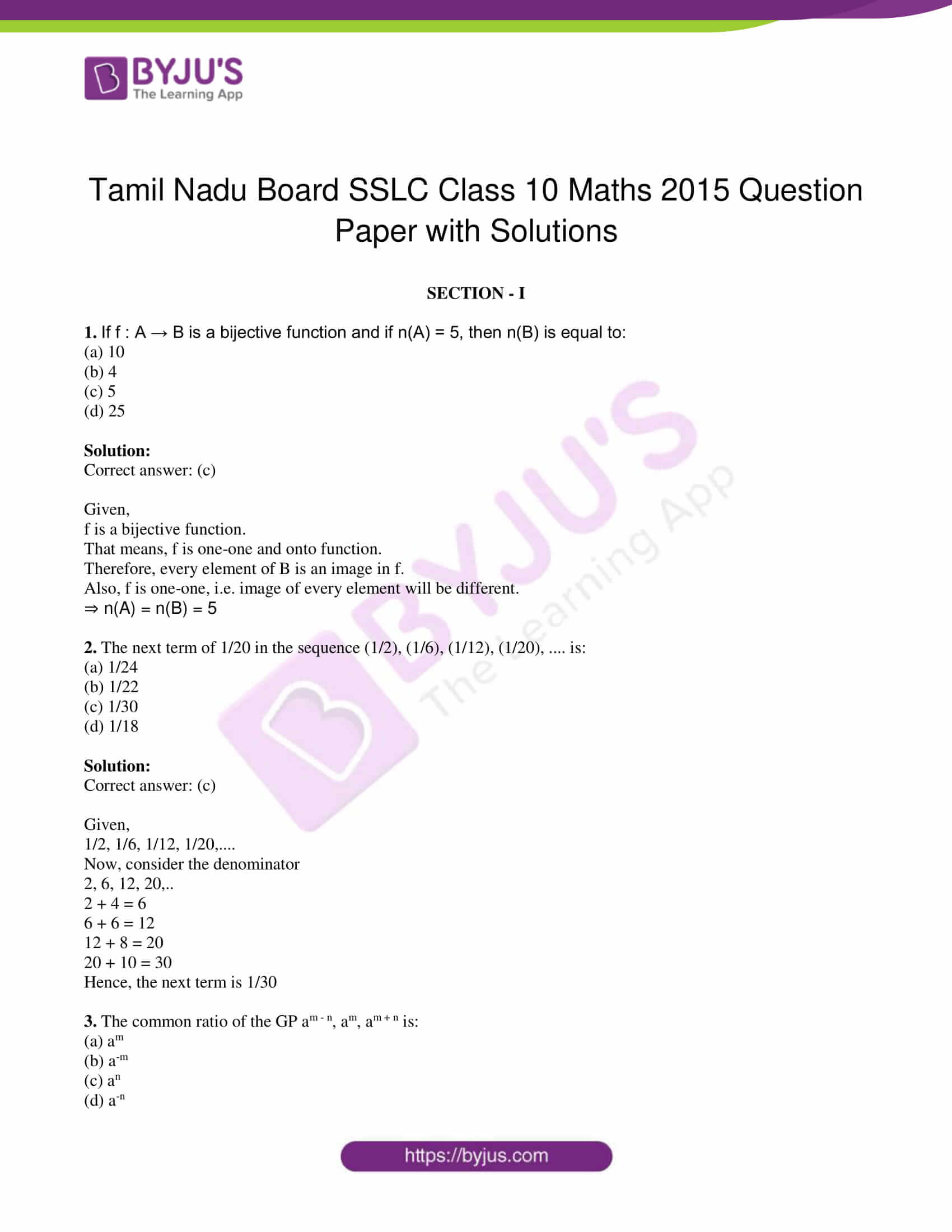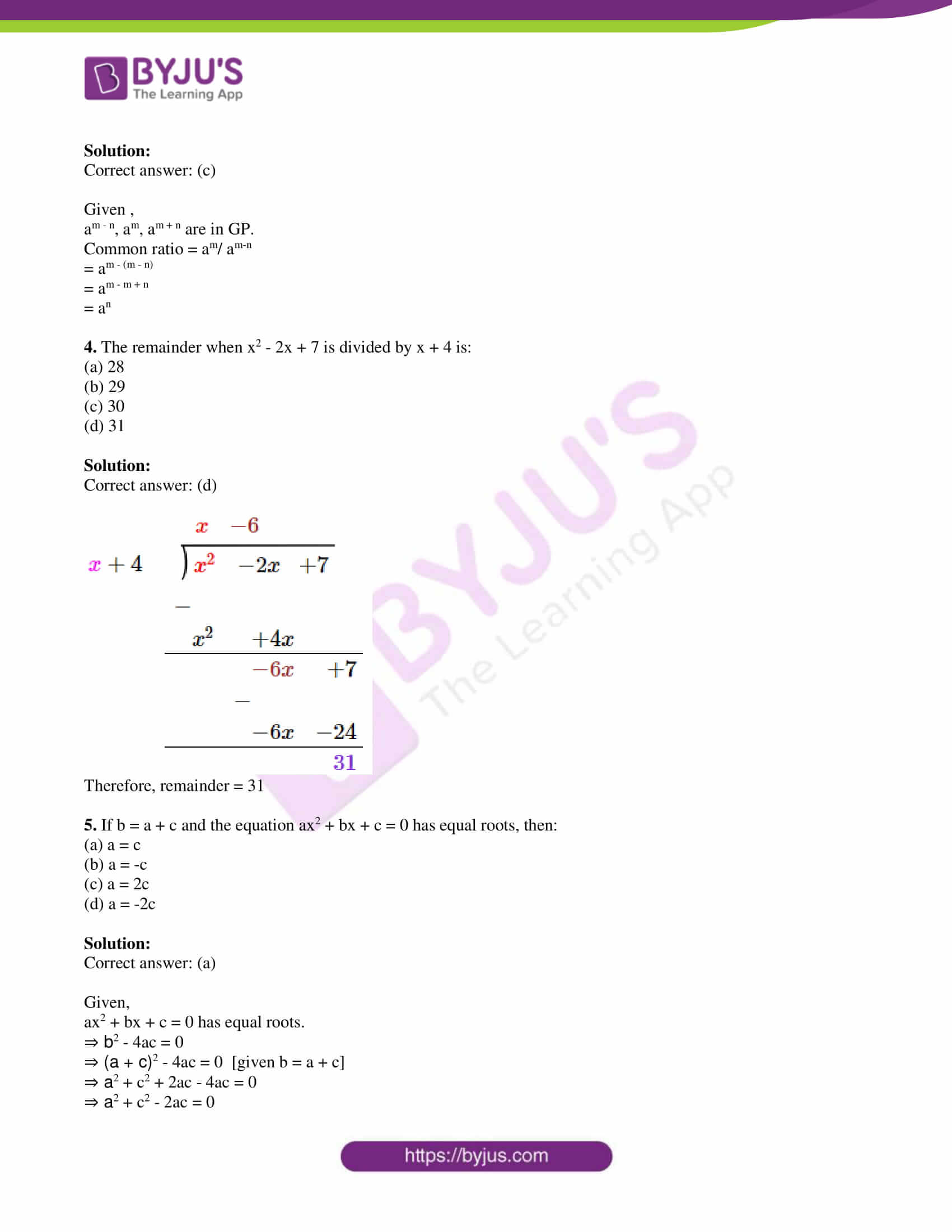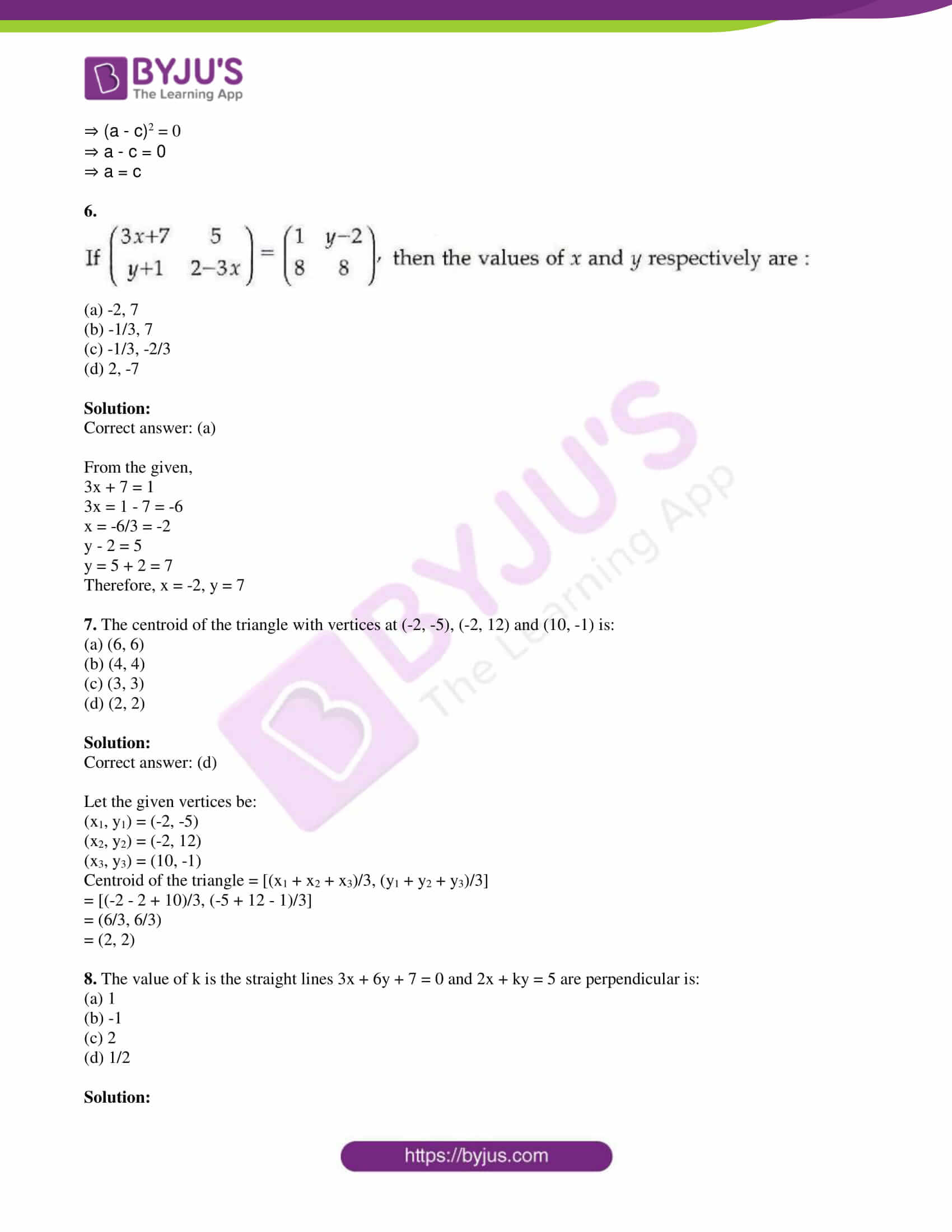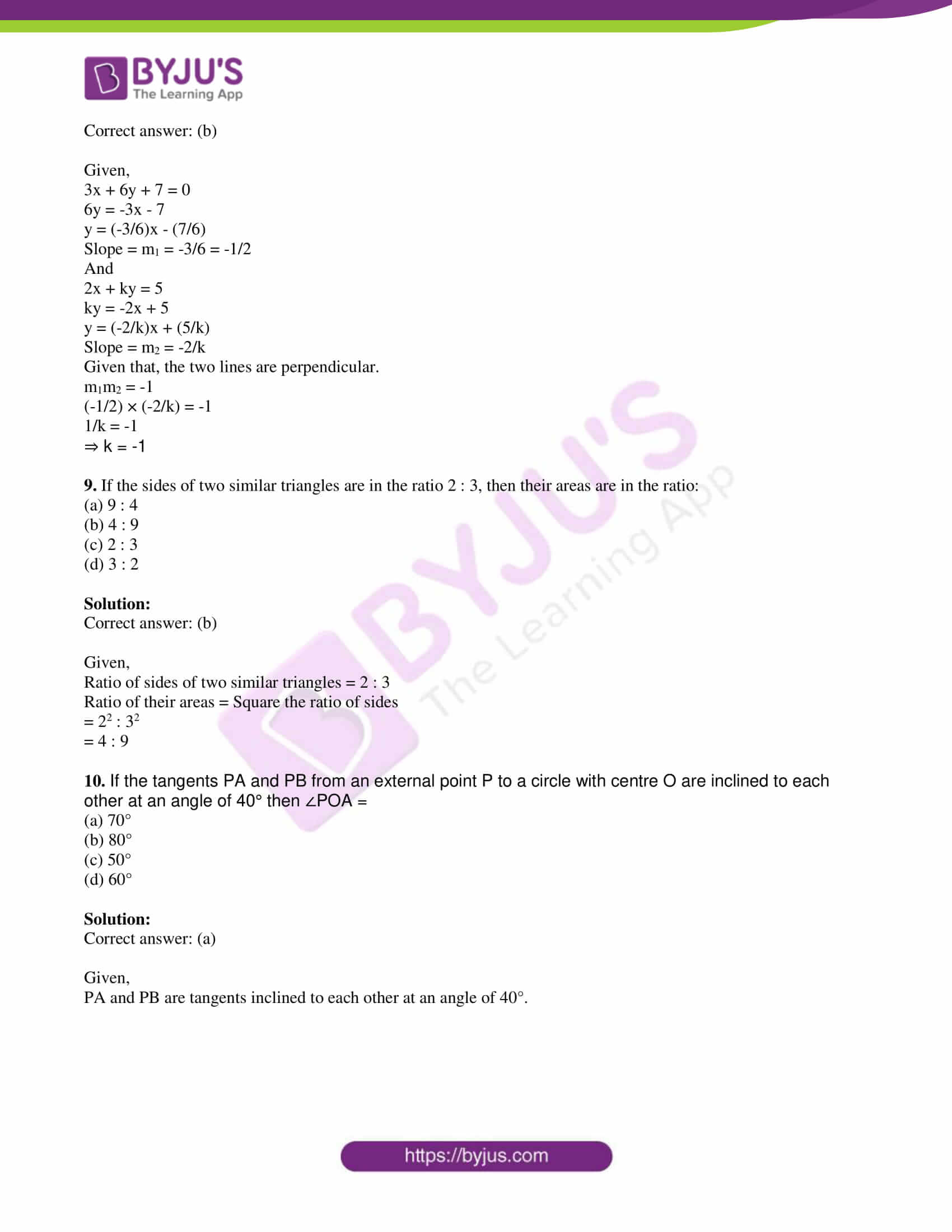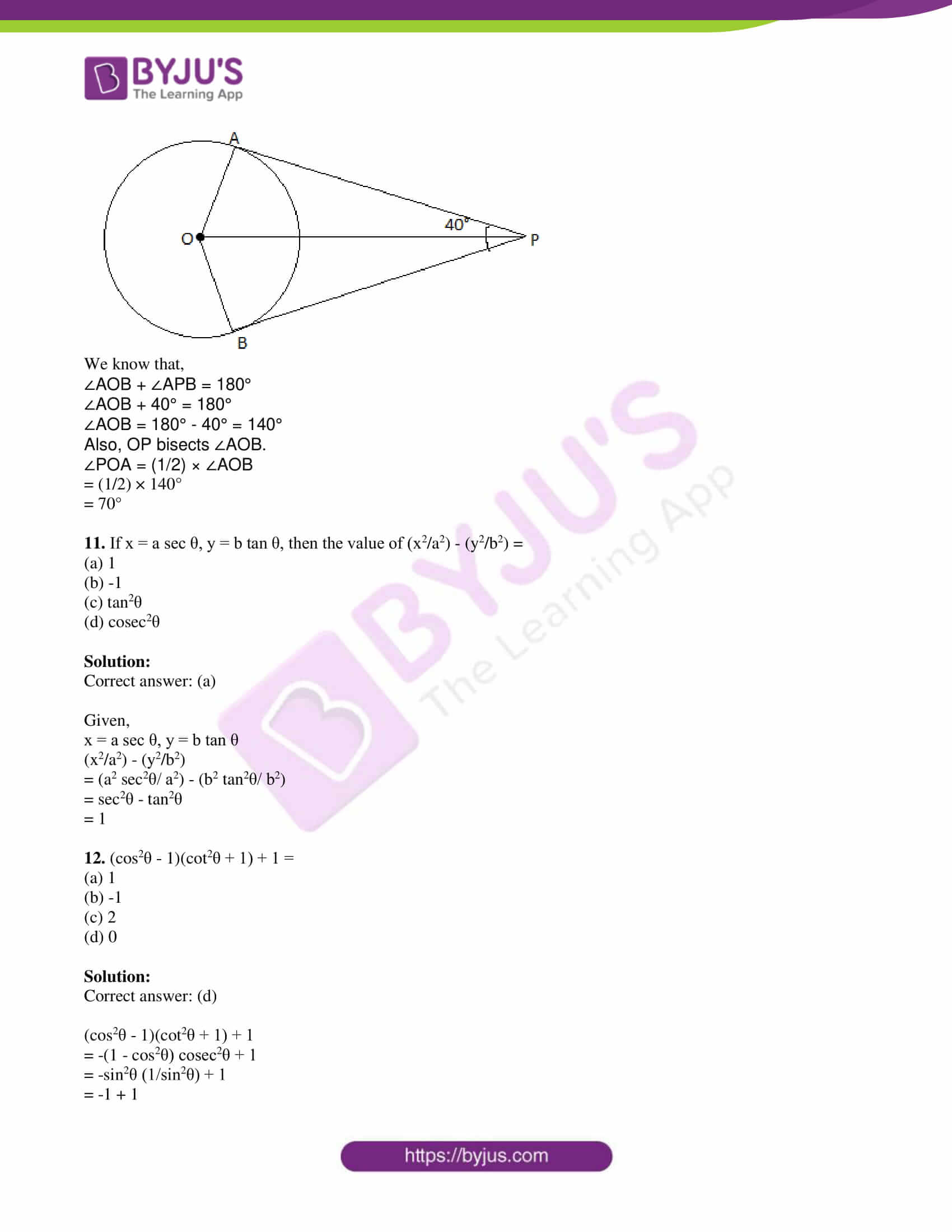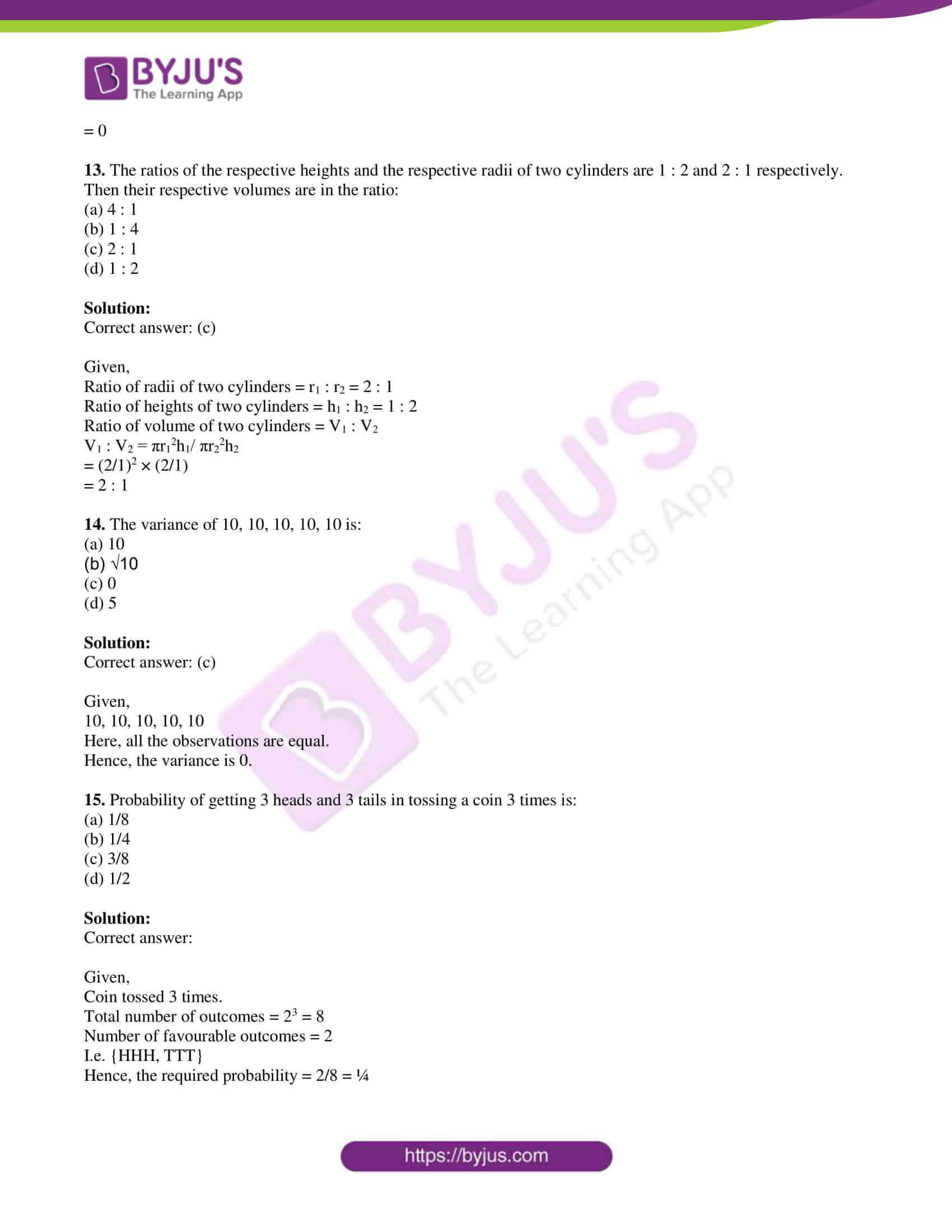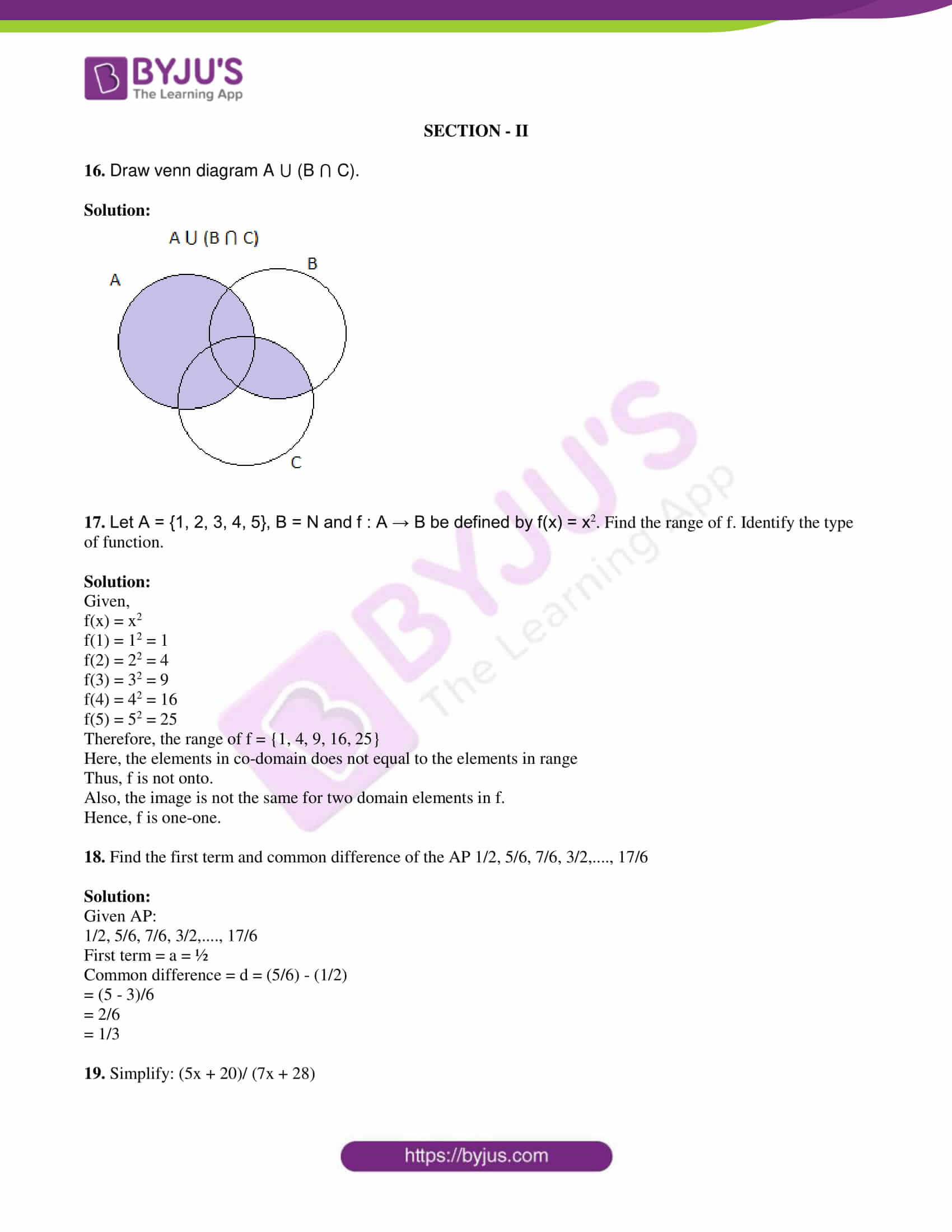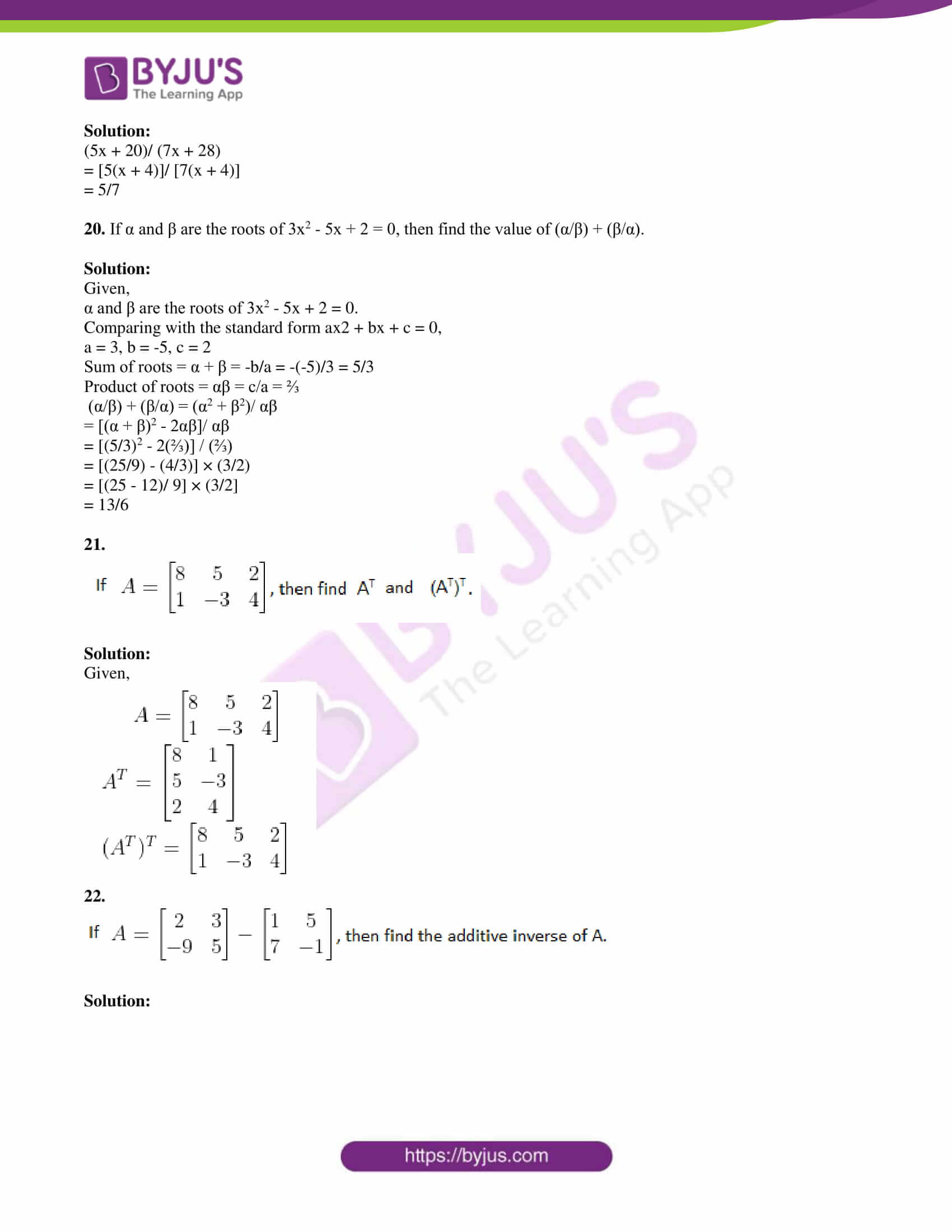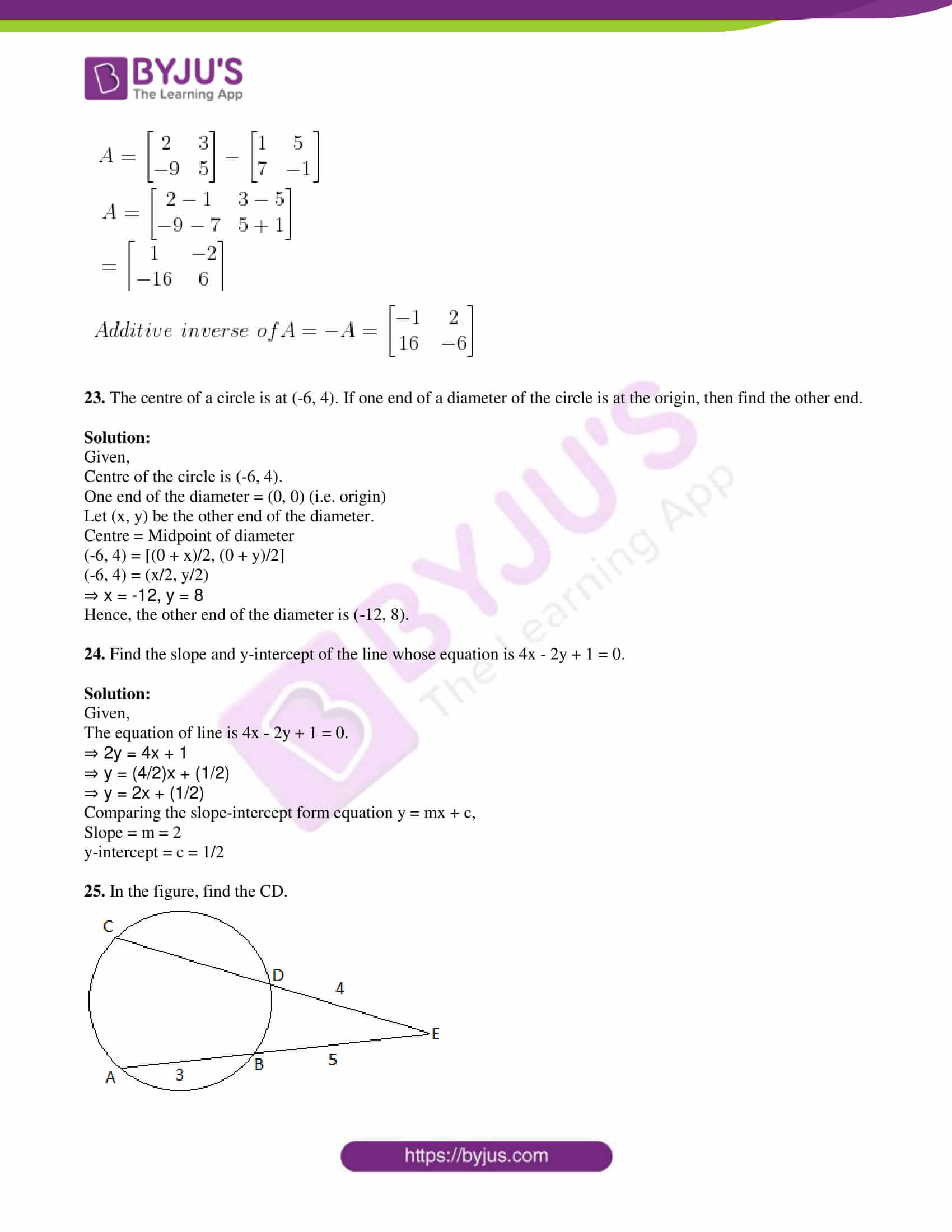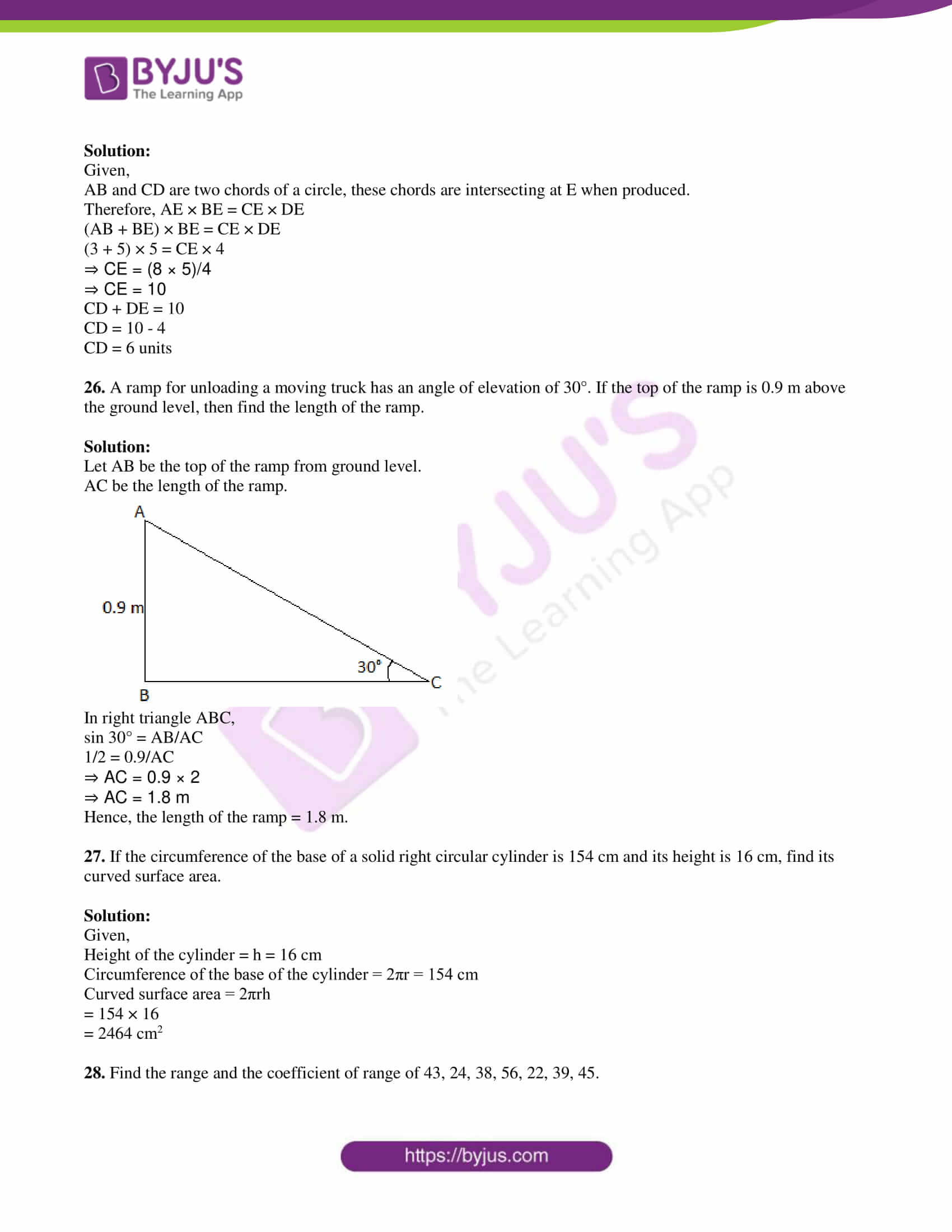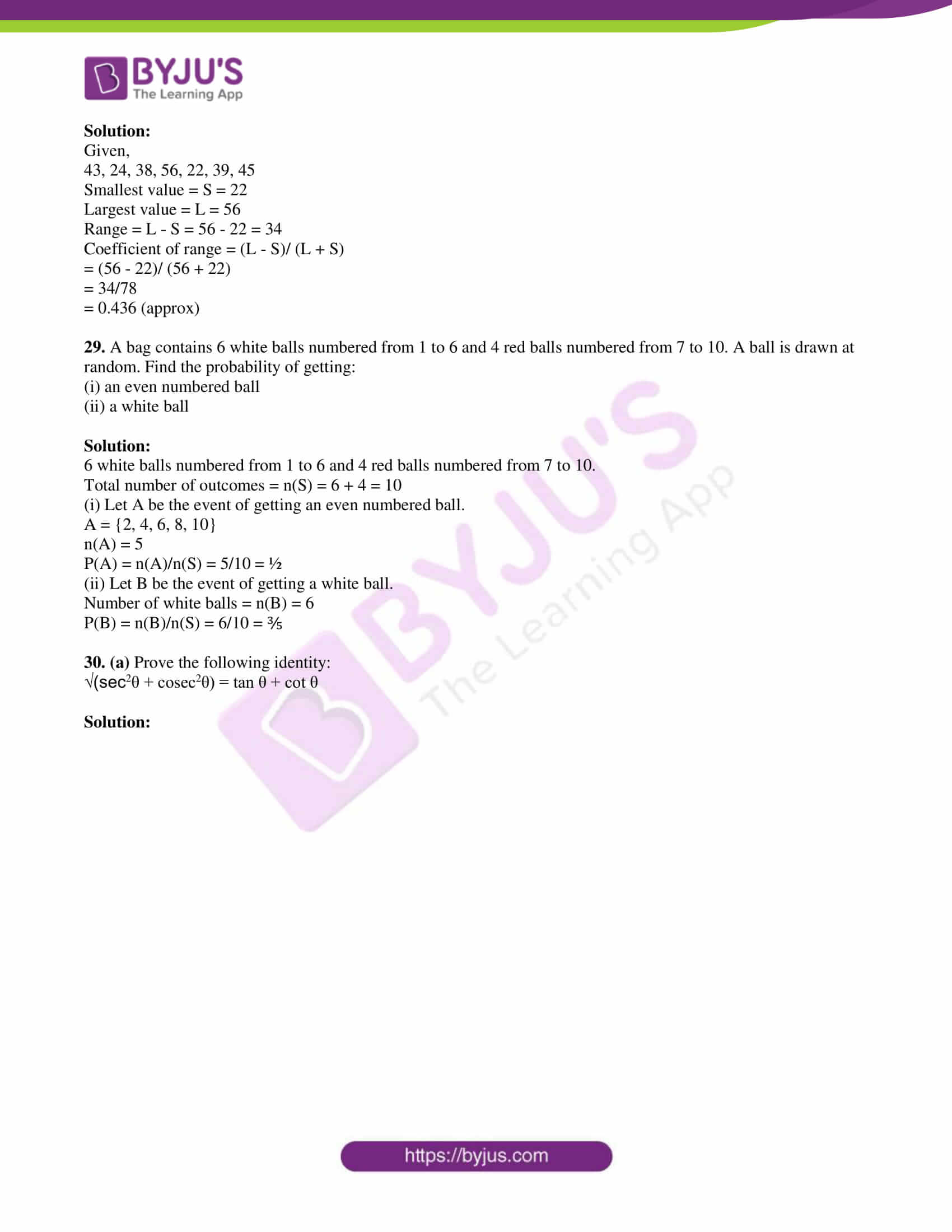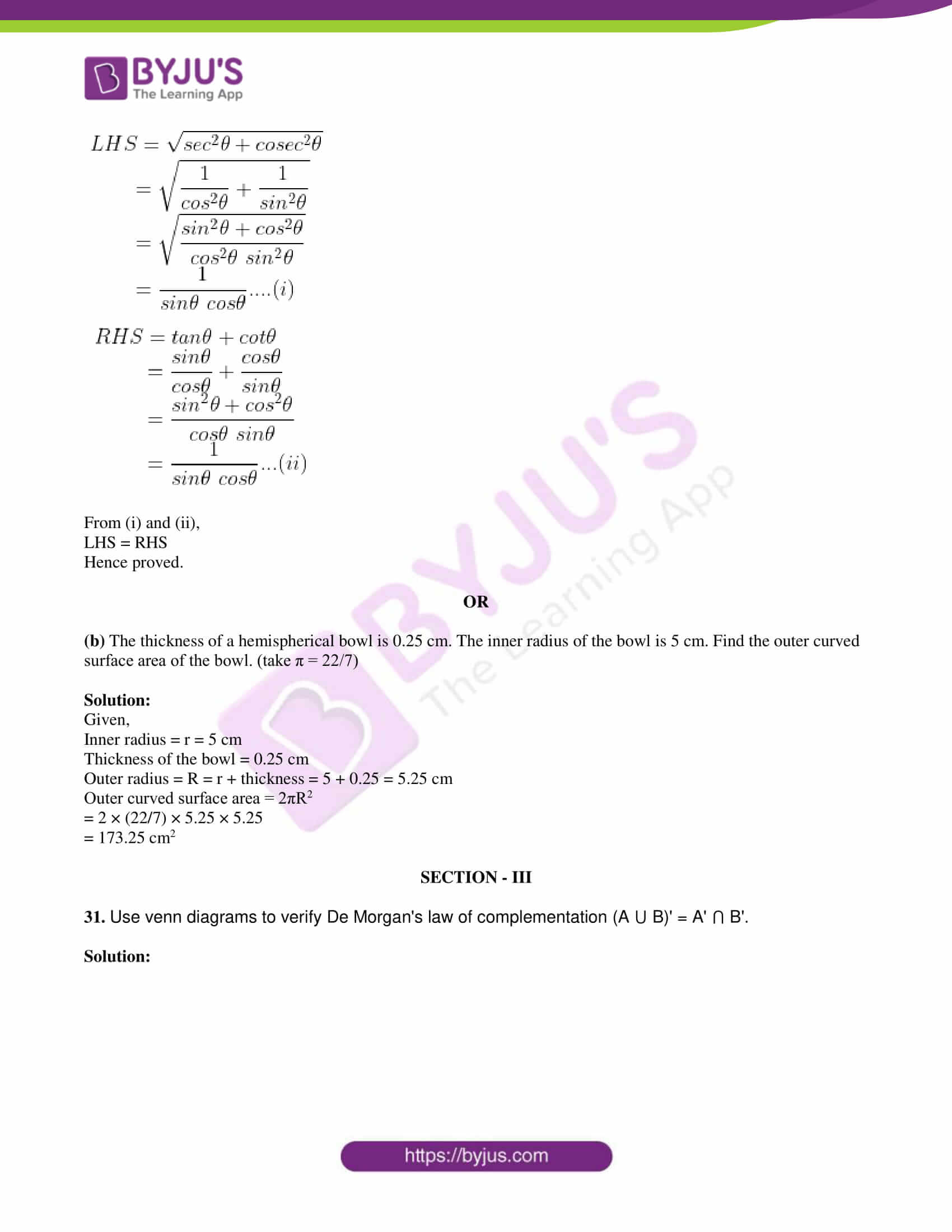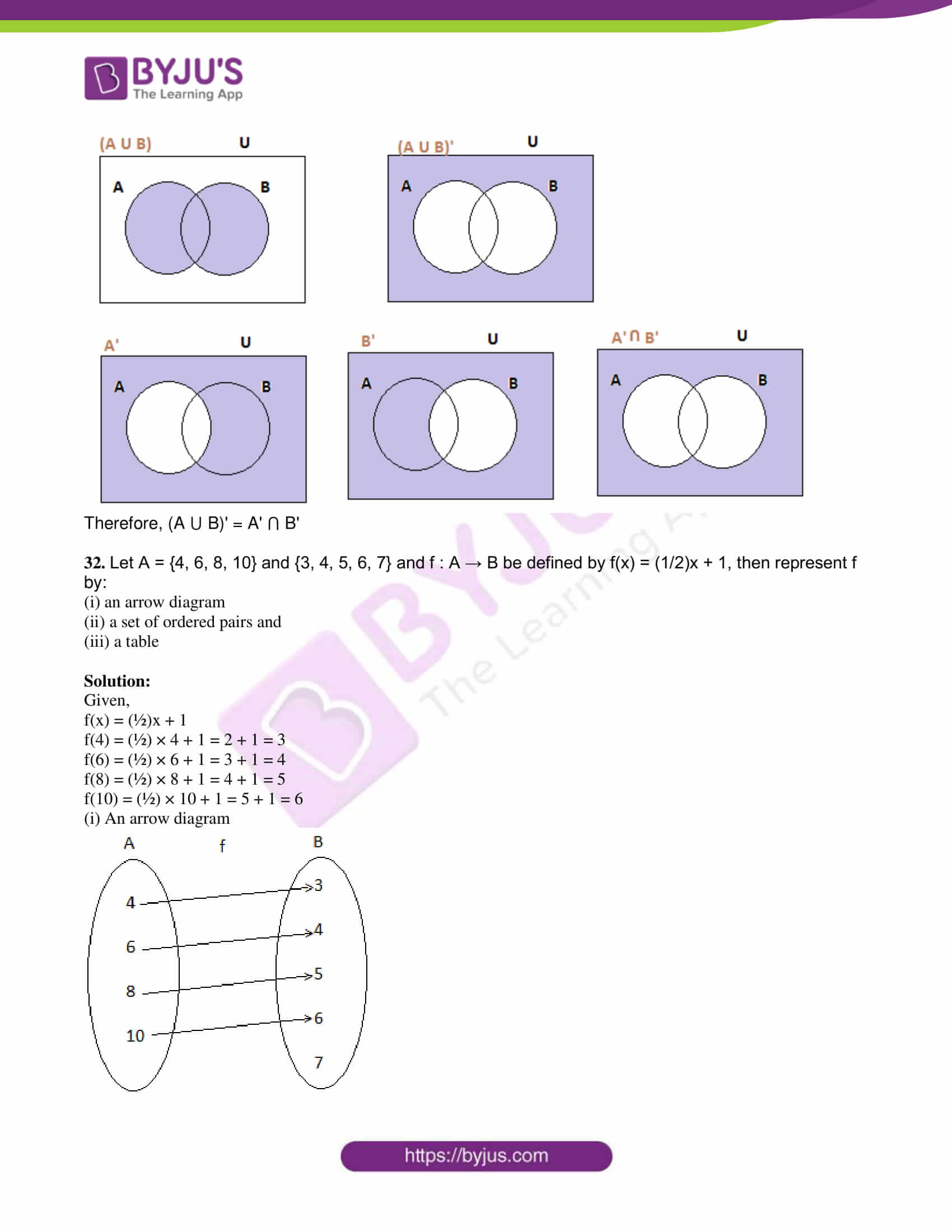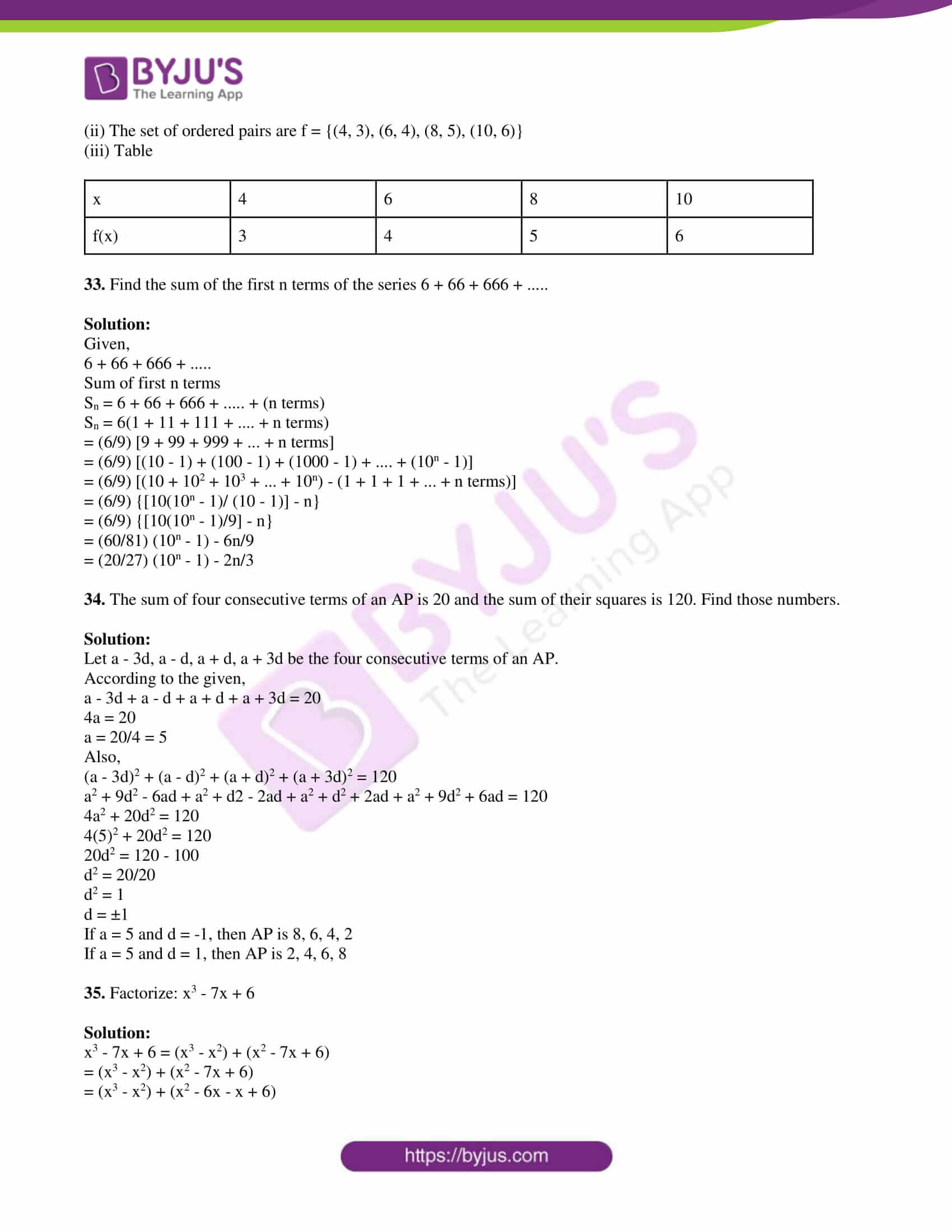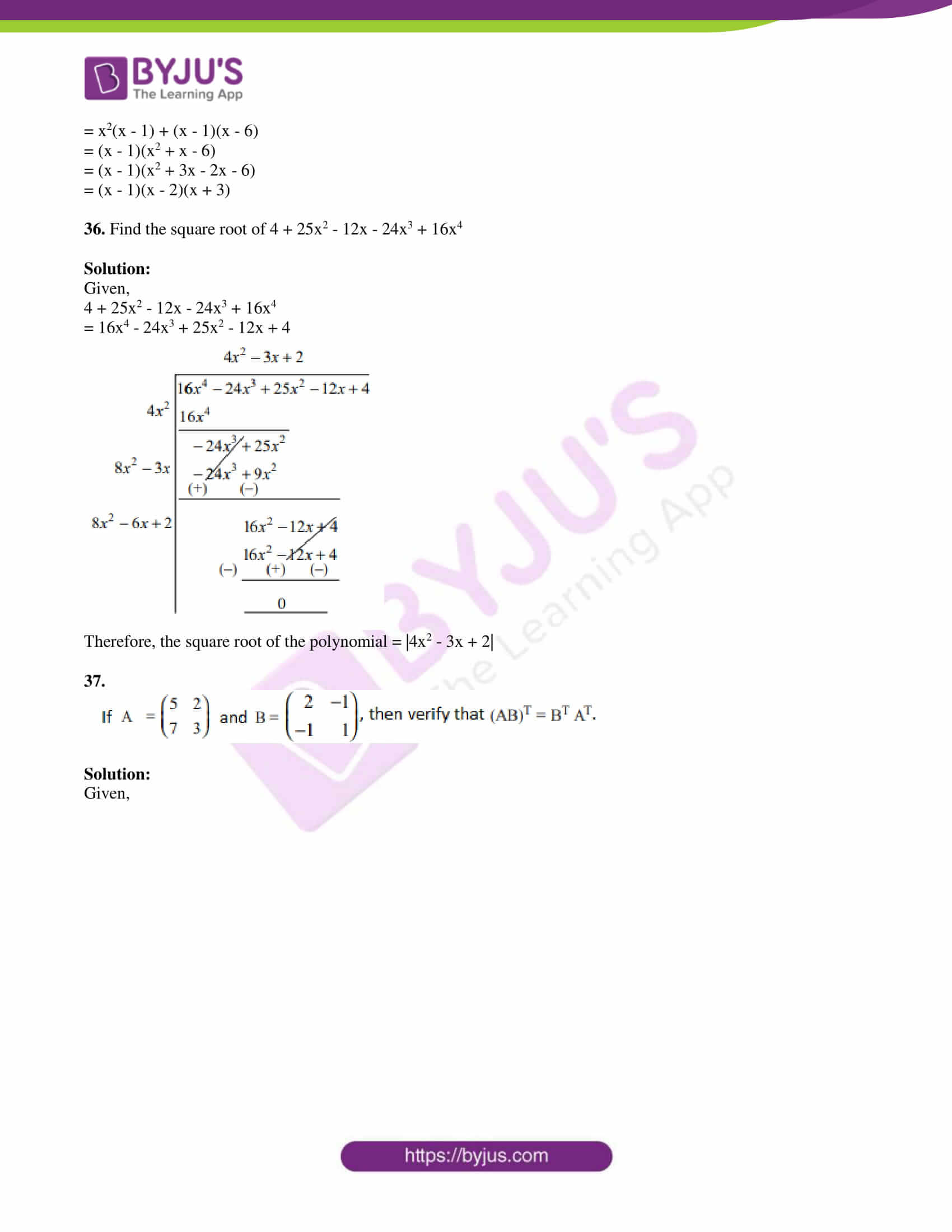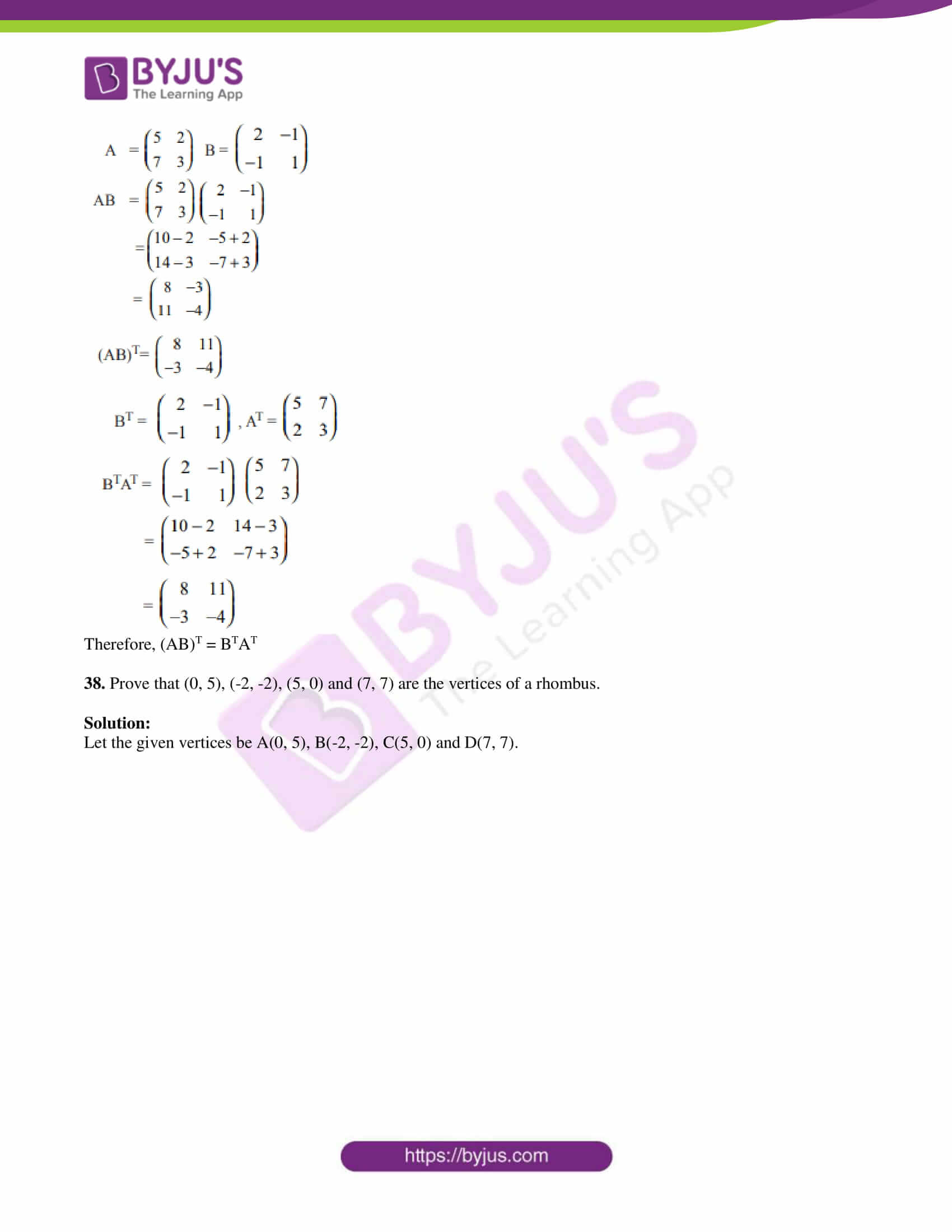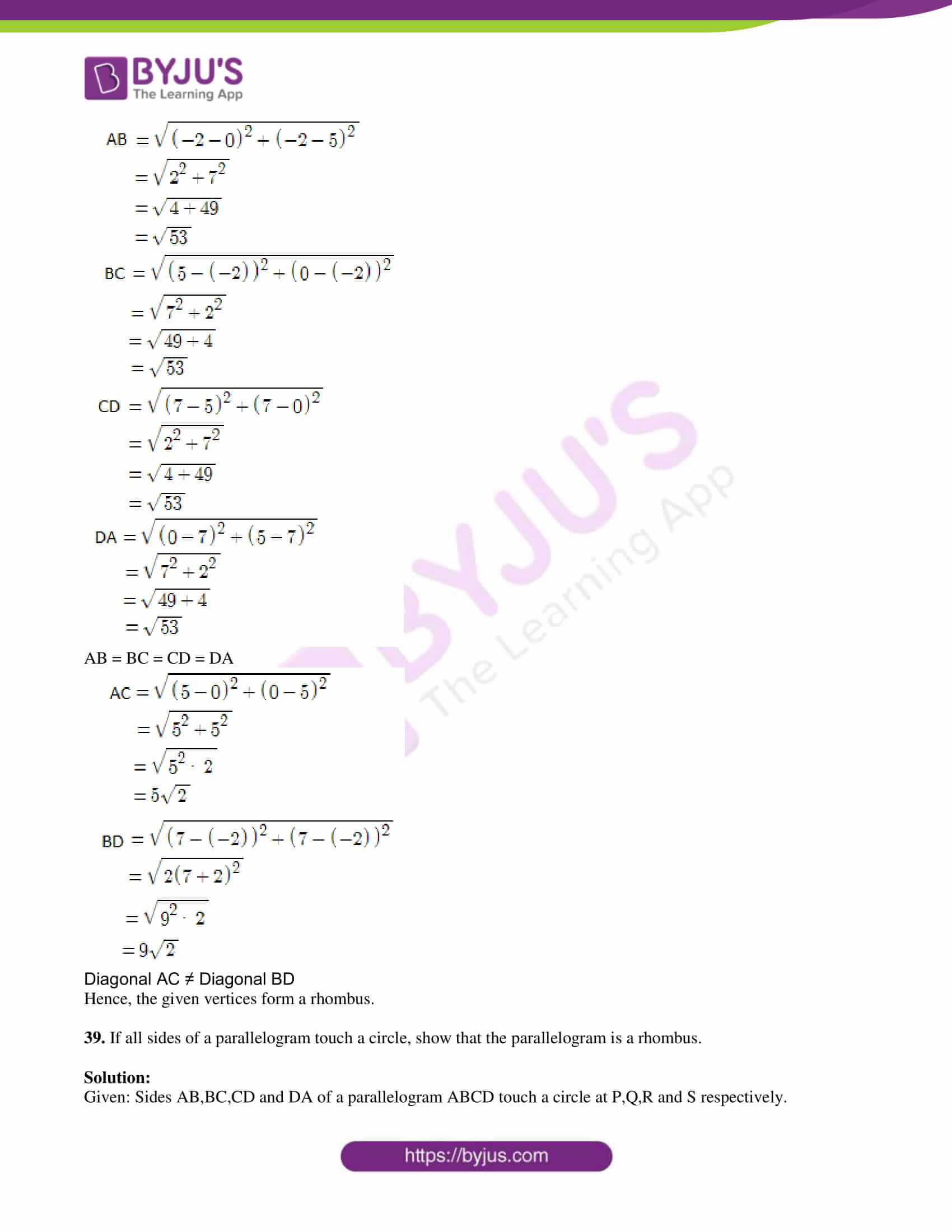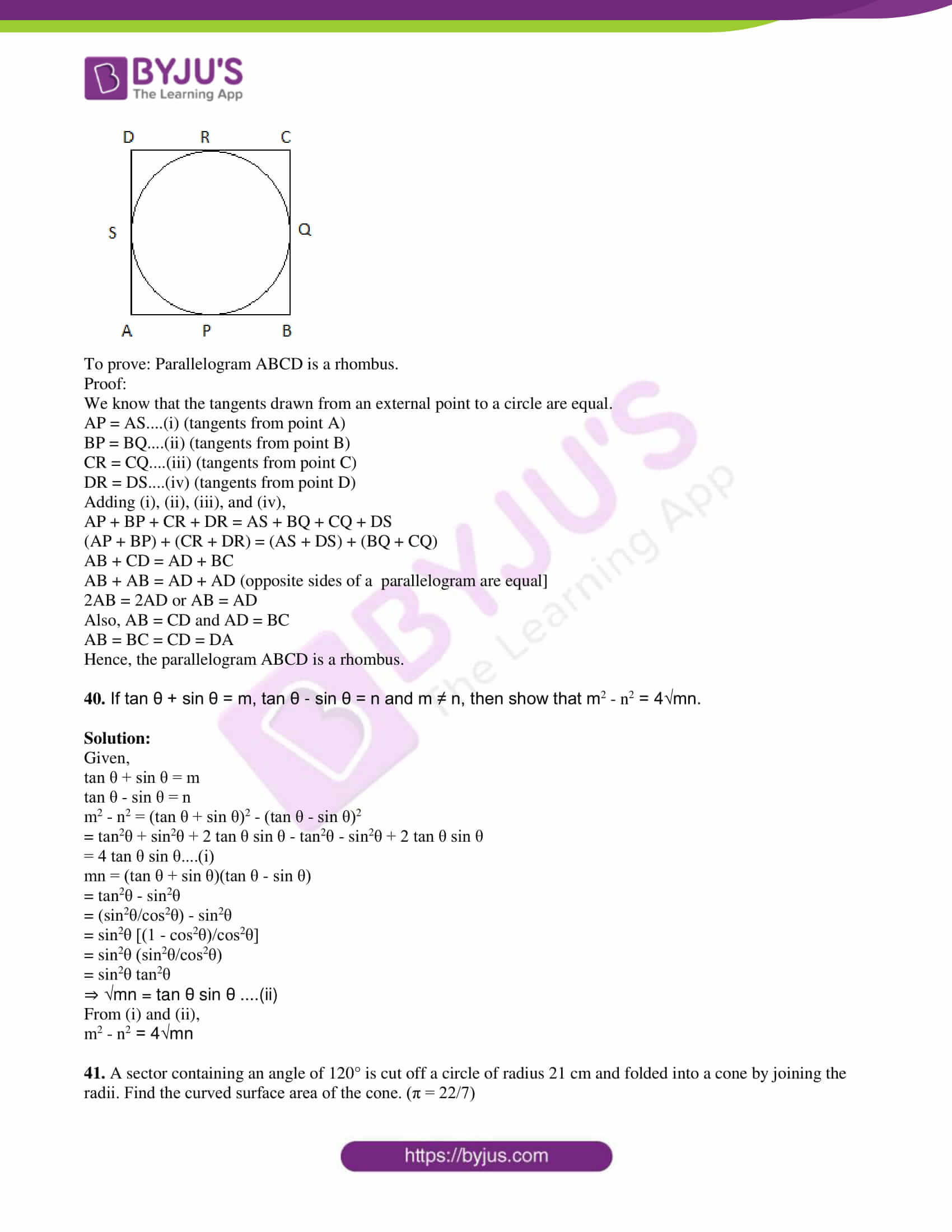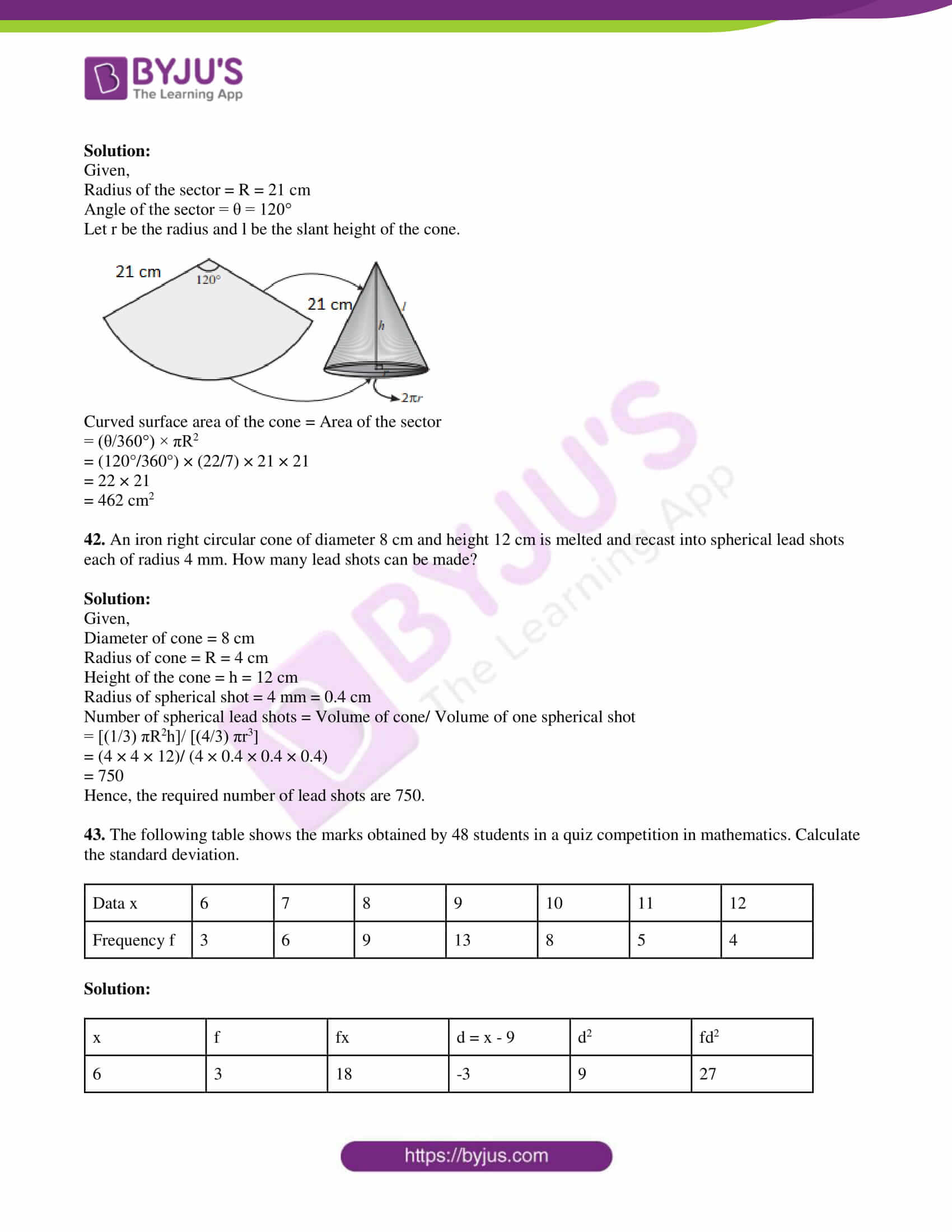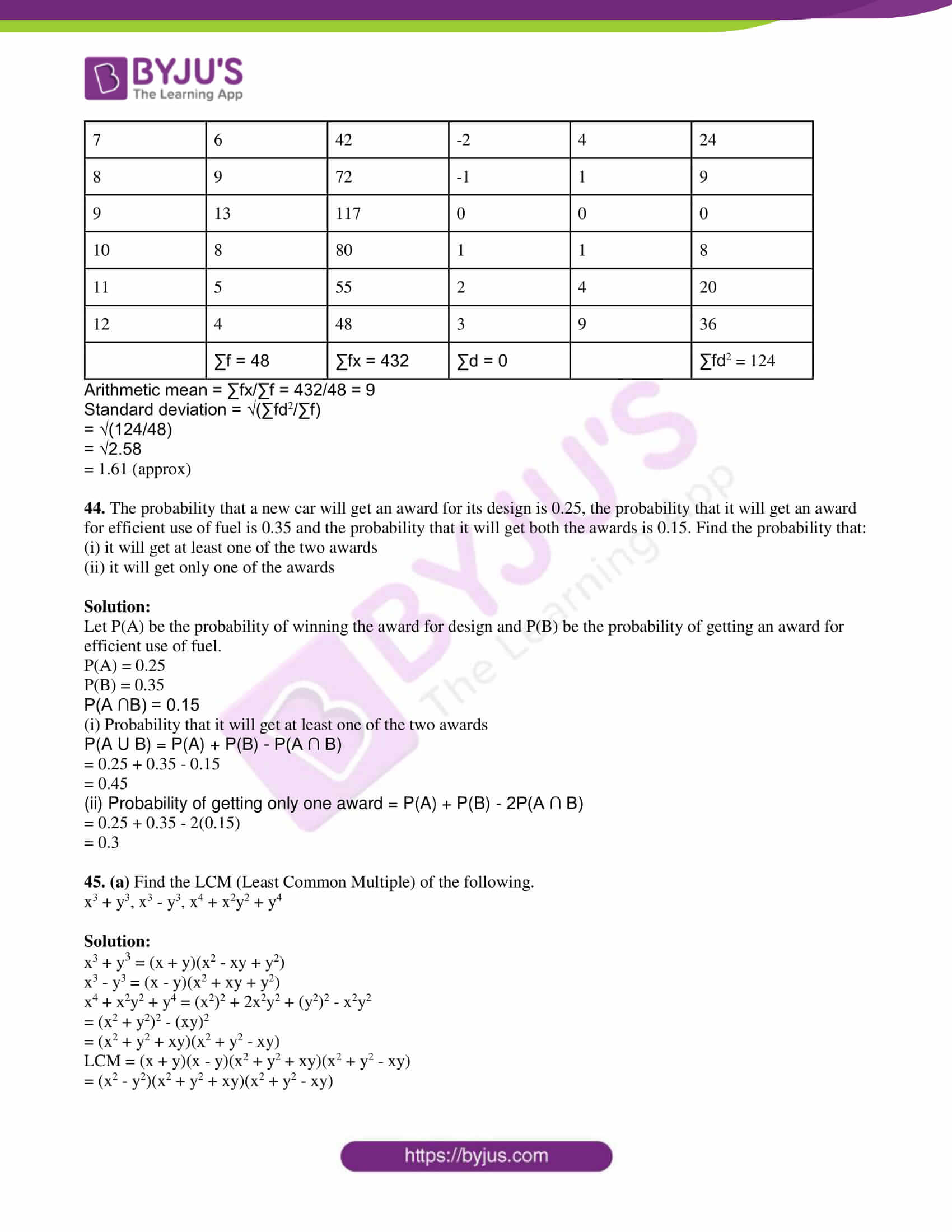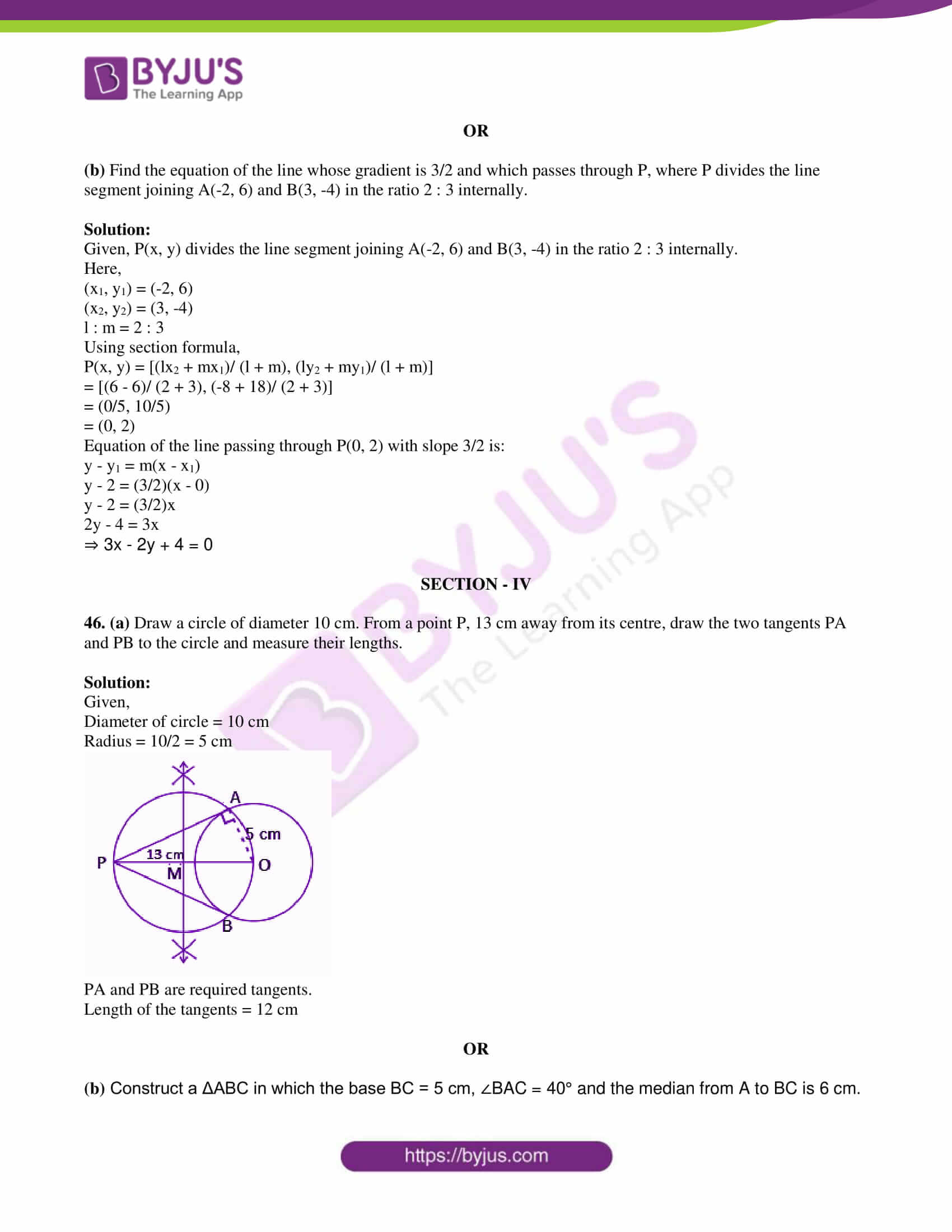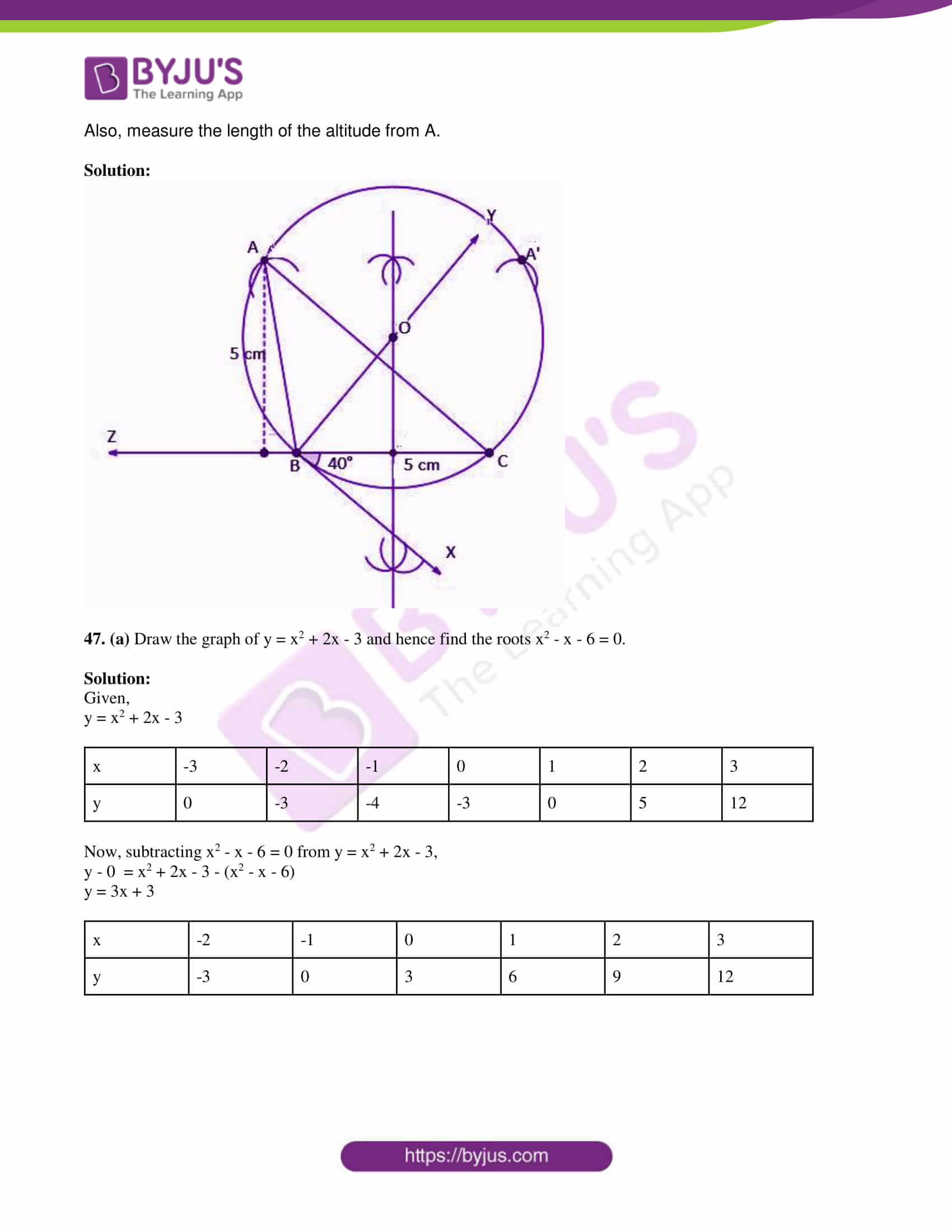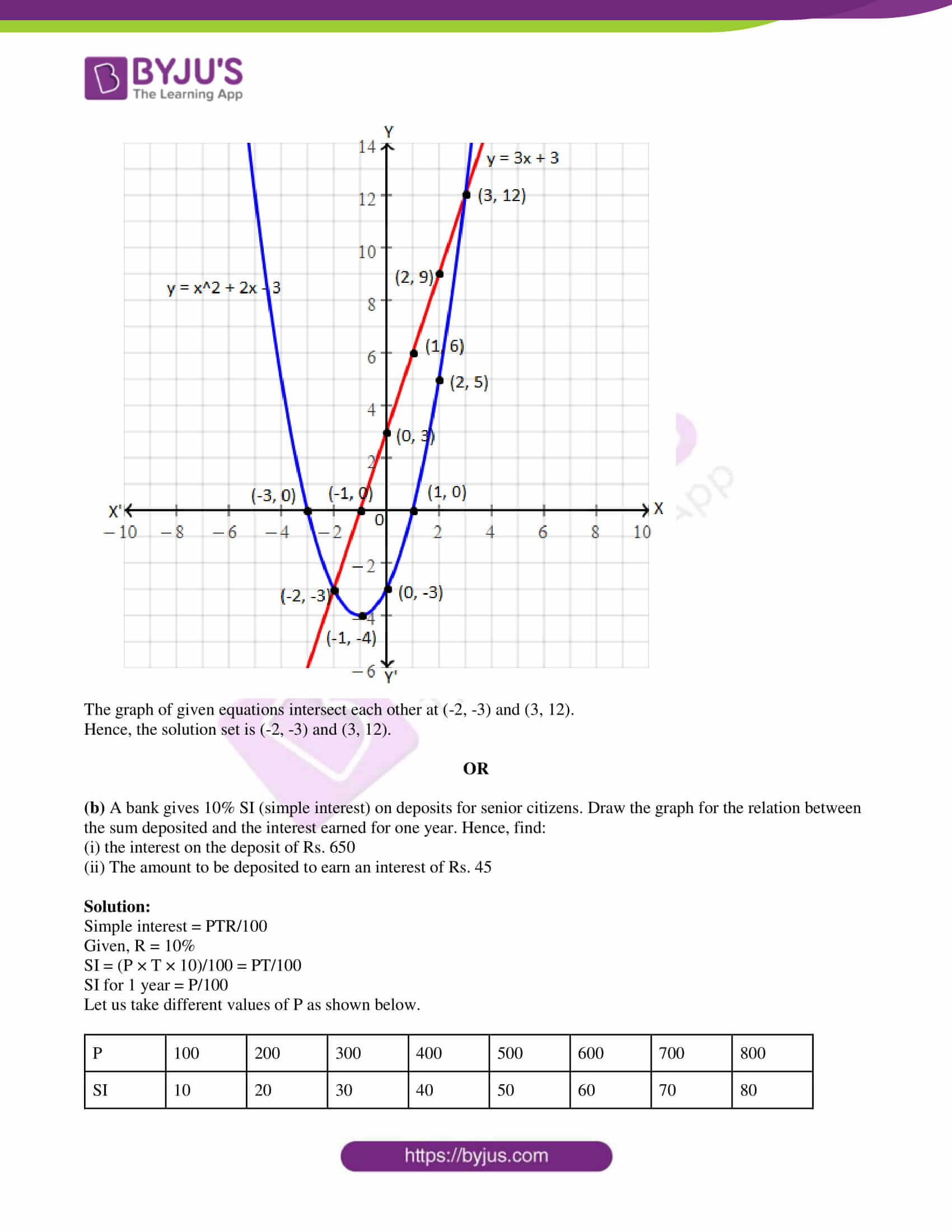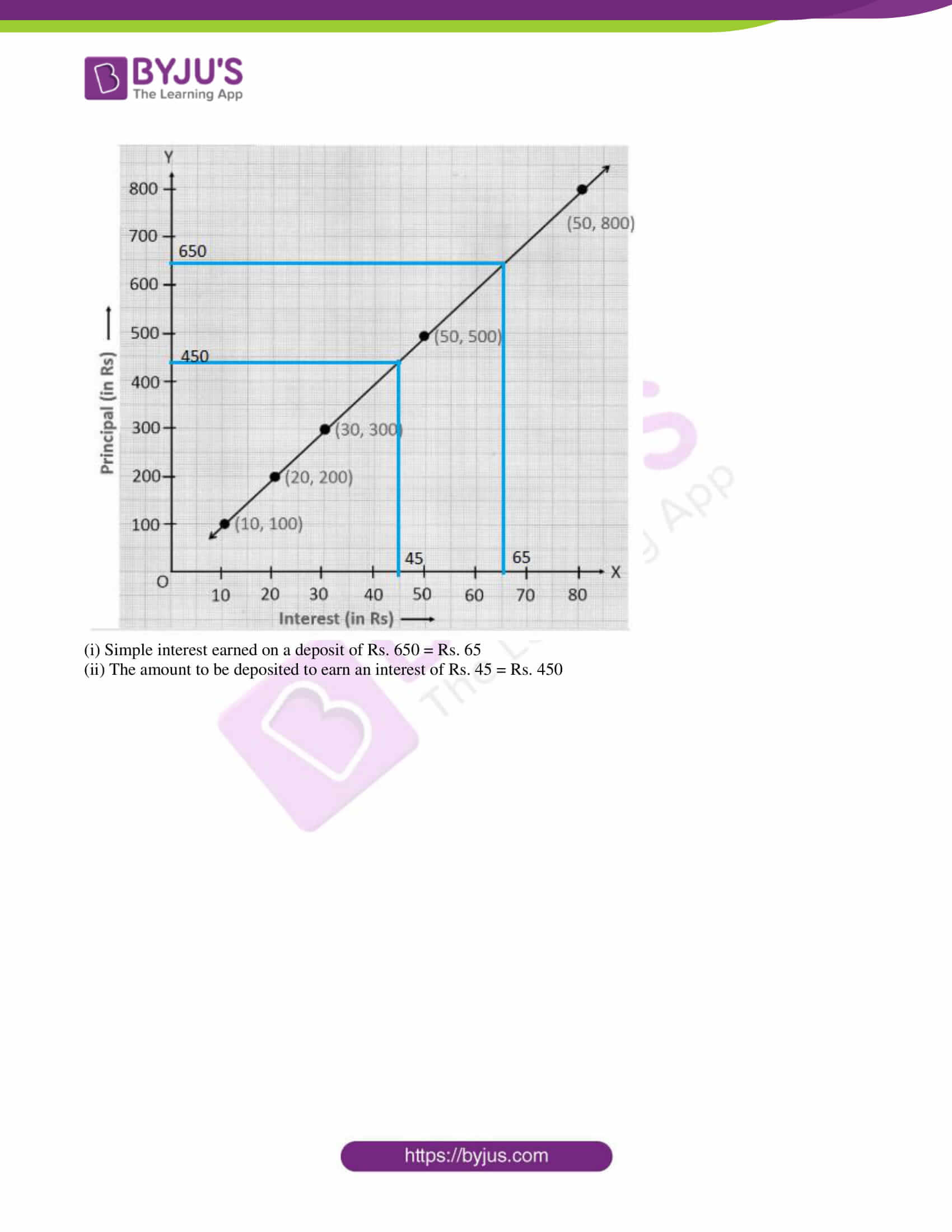SECTION – I

1. If f : A → B is a bijective function and if n(A) = 5, then n(B) is equal to:

(a) 10

(b) 4

(c) 5

(d) 25

Solution:

Given,

f is a bijective function.

That means, f is one-one and onto function.

Therefore, every element of B is an image in f.

Also, f is one-one, i.e. image of every element will be different.

⇒ n(A) = n(B) = 5

2. The next term of 1/20 in the sequence (1/2), (1/6), (1/12), (1/20), …. is:

(a) 1/24

(b) 1/22

(c) 1/30

(d) 1/18

Solution:

Given,

1/2, 1/6, 1/12, 1/20,….

Now, consider the denominator

2, 6, 12, 20,..

2 + 4 = 6

6 + 6 = 12

12 + 8 = 20

20 + 10 = 30

Hence, the next term is 1/30

3. The common ratio of the GP am – n, am, am + n is:

(a) am

(b) a-m

(c) an

(d) a-n

Solution:

Given,

am – n, am, am + n are in GP.

Common ratio = am/ am-n

= am – (m – n)

= am – m + n

= an

4. The remainder when x2 – 2x + 7 is divided by x + 4 is:

(a) 28

(b) 29

(c) 30

(d) 31

Solution: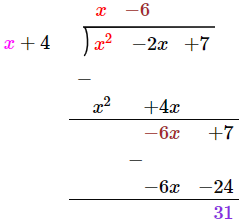Therefore, the remainder = 31

5. If b = a + c and the equation ax2 + bx + c = 0 has equal roots, then:

(a) a = c

(b) a = -c

(c) a = 2c

(d) a = -2c

Solution:

Given,

ax2 + bx + c = 0 has equal roots.

⇒ b2 – 4ac = 0

⇒ (a + c)2 – 4ac = 0 [given b = a + c]

⇒ a2 + c2 + 2ac – 4ac = 0

⇒ a2 + c2 – 2ac = 0

⇒ (a – c)2 = 0

⇒ a – c = 0

⇒ a = c

6.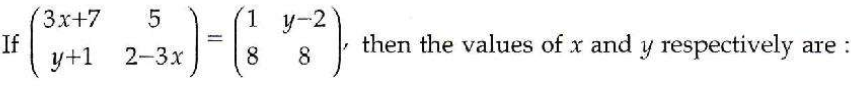(a) -2, 7

(b) -1/3, 7

(c) -1/3, -2/3

(d) 2, -7

Solution:

From the given,

3x + 7 = 1

3x = 1 – 7 = -6

x = -6/3 = -2

y – 2 = 5

y = 5 + 2 = 7

Therefore, x = -2, y = 7

7. The centroid of the triangle with vertices at (-2, -5), (-2, 12) and (10, -1) is:

(a) (6, 6)

(b) (4, 4)

(c) (3, 3)

(d) (2, 2)

Solution:

Let the given vertices be:

(x1, y1) = (-2, -5)

(x2, y2) = (-2, 12)

(x3, y3) = (10, -1)

Centroid of the triangle = [(x1 + x2 + x3)/3, (y1 + y2 + y3)/3]

= [(-2 – 2 + 10)/3, (-5 + 12 – 1)/3]

= (6/3, 6/3)

= (2, 2)

8. The value of k is the straight lines 3x + 6y + 7 = 0 and 2x + ky = 5 are perpendicular is:

(a) 1

(b) -1

(c) 2

(d) 1/2

Solution:

Given,

3x + 6y + 7 = 0

6y = -3x – 7

y = (-3/6)x – (7/6)

Slope = m1 = -3/6 = -1/2

And

2x + ky = 5

ky = -2x + 5

y = (-2/k)x + (5/k)

Slope = m2 = -2/k

Given that, the two lines are perpendicular.

m1m2 = -1

(-1/2) × (-2/k) = -1

1/k = -1

⇒ k = -1

9. If the sides of two similar triangles are in the ratio 2 : 3, then their areas are in the ratio:

(a) 9 : 4

(b) 4 : 9

(c) 2 : 3

(d) 3 : 2

Solution:

Given,

Ratio of sides of two similar triangles = 2 : 3

Ratio of their areas = Square the ratio of sides

= 22 : 32

= 4 : 9

10. If the tangents PA and PB from an external point P to a circle with centre O are inclined to each other at an angle of 40° then ∠POA =

(a) 70°

(b) 80°

(c) 50°

(d) 60°

Solution:

Given,

PA and PB are tangents inclined to each other at an angle of 40°.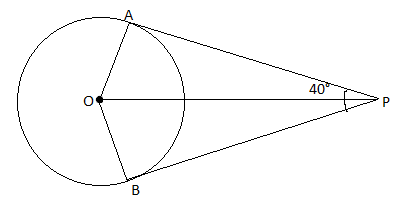We know that,

∠AOB + ∠APB = 180°

∠AOB + 40° = 180°

∠AOB = 180° – 40° = 140°

Also, OP bisects ∠AOB.

∠POA = (1/2) × ∠AOB

= (1/2) × 140°

= 70°

11. If x = a sec θ, y = b tan θ, then the value of (x2/a2) – (y2/b2) =

(a) 1

(b) -1

(c) tan2θ

(d) cosec2θ

Solution:

Given,

x = a sec θ, y = b tan θ

(x2/a2) – (y2/b2)

= (a2 sec2θ/ a2) – (b2 tan2θ/ b2)

= sec2θ – tan2θ

= 1

12. (cos2θ – 1)(cot2θ + 1) + 1 =

(a) 1

(b) -1

(c) 2

(d) 0

Solution:

(cos2θ – 1)(cot2θ + 1) + 1

= -(1 – cos2θ) cosec2θ + 1

= -sin2θ (1/sin2θ) + 1

= -1 + 1

= 0

13. The ratios of the respective heights and the respective radii of two cylinders are 1 : 2 and 2 : 1 respectively. Then their respective volumes are in the ratio:

(a) 4 : 1

(b) 1 : 4

(c) 2 : 1

(d) 1 : 2

Solution:

Given,

Ratio of radii of two cylinders = r1 : r2 = 2 : 1

Ratio of heights of two cylinders = h1 : h2 = 1 : 2

Ratio of volume of two cylinders = V1 : V2

V1 : V2 = πr12h1/ πr22h2

= (2/1)2 × (2/1)

= 2 : 1

14. The variance of 10, 10, 10, 10, 10 is:

(a) 10

(b) √10

(c) 0

(d) 5

Solution:

Given,

10, 10, 10, 10, 10

Here, all the observations are equal.

Hence, the variance is 0.

15. Probability of getting 3 heads and 3 tails in tossing a coin 3 times is:

(a) 1/8

(b) 1/4

(c) 3/8

(d) 1/2

Solution:

Given,

Coin tossed 3 times.

Total number of outcomes = 23 = 8

Number of favourable outcomes = 2

I.e. {HHH, TTT}

Hence, the required probability = 2/8 = ¼

SECTION – II

16. Draw Venn diagram A ⋃ (B ⋂ C).

Solution: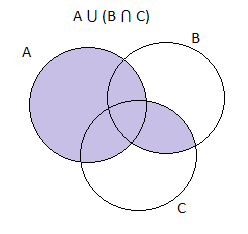17. Let A = {1, 2, 3, 4, 5}, B = N and f : A → B be defined by f(x) = x2. Find the range of f. Identify the type of function.

Solution:

Given,

f(x) = x2

f(1) = 12 = 1

f(2) = 22 = 4

f(3) = 32 = 9

f(4) = 42 = 16

f(5) = 52 = 25

Therefore, the range of f = {1, 4, 9, 16, 25}

Here, the elements in co-domain does not equal to the elements in range

Thus, f is not onto.

Also, the image is not the same for two domain elements in f.

Hence, f is one-one.

18. Find the first term and common difference of the AP 1/2, 5/6, 7/6, 3/2,…., 17/6

Solution:

Given AP:

1/2, 5/6, 7/6, 3/2,…., 17/6

First term = a = ½

Common difference = d = (5/6) – (1/2)

= (5 – 3)/6

= 2/6

= 1/3

19. Simplify: (5x + 20)/ (7x + 28)

Solution:

(5x + 20)/ (7x + 28)

= [5(x + 4)]/ [7(x + 4)]

= 5/7

20. If α and β are the roots of 3x2 – 5x + 2 = 0, then find the value of (α/β) + (β/α).

Solution:

Given,

α and β are the roots of 3x2 – 5x + 2 = 0.

Comparing with the standard form ax2 + bx + c = 0,

a = 3, b = -5, c = 2

Sum of roots = α + β = -b/a = -(-5)/3 = 5/3

Product of roots = αβ = c/a = ⅔

(α/β) + (β/α) = (α2 + β2)/ αβ

= [(α + β)2 – 2αβ]/ αβ

= [(5/3)2 – 2(⅔)] / (⅔)

= [(25/9) – (4/3)] × (3/2)

= [(25 – 12)/ 9] × (3/2]

= 13/6

21.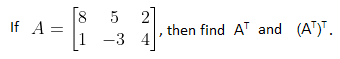Solution:

Given,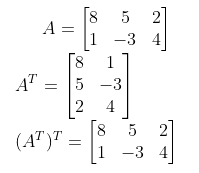22.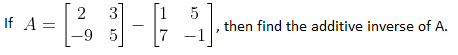Solution: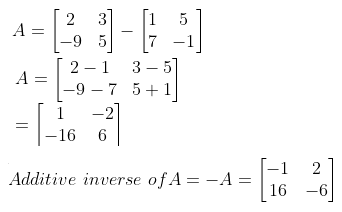23. The centre of a circle is at (-6, 4). If one end of a diameter of the circle is at the origin, then find the other end.

Solution:

Given,

Centre of the circle is (-6, 4).

One end of the diameter = (0, 0) (i.e. origin)

Let (x, y) be the other end of the diameter.

Centre = Midpoint of diameter

(-6, 4) = [(0 + x)/2, (0 + y)/2]

(-6, 4) = (x/2, y/2)

⇒ x = -12, y = 8

Hence, the other end of the diameter is (-12, 8).

24. Find the slope and y-intercept of the line whose equation is 4x – 2y + 1 = 0.

Solution:

Given,

The equation of line is 4x – 2y + 1 = 0.

⇒ 2y = 4x + 1

⇒ y = (4/2)x + (1/2)

⇒ y = 2x + (1/2)

Comparing the slope-intercept form equation y = mx + c,

Slope = m = 2

y-intercept = c = 1/2

25. In the figure, find the CD.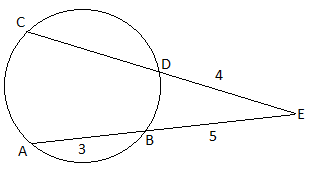Solution:

Given,

AB and CD are two chords of a circle, these chords are intersecting at E when produced.

Therefore, AE × BE = CE × DE

(AB + BE) × BE = CE × DE

(3 + 5) × 5 = CE × 4

⇒ CE = (8 × 5)/4

⇒ CE = 10

CD + DE = 10

CD = 10 – 4

CD = 6 units

26. A ramp for unloading a moving truck has an angle of elevation of 30°. If the top of the ramp is 0.9 m above the ground level, then find the length of the ramp.

Solution:

Let AB be the top of the ramp from ground level.

AC be the length of the ramp.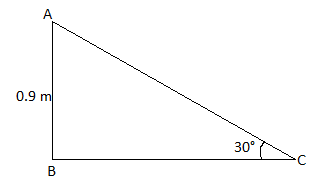In right triangle ABC,

sin 30° = AB/AC

1/2 = 0.9/AC

⇒ AC = 0.9 × 2

⇒ AC = 1.8 m

Hence, the length of the ramp = 1.8 m.

27. If the circumference of the base of a solid right circular cylinder is 154 cm and its height is 16 cm, find its curved surface area.

Solution:

Given,

Height of the cylinder = h = 16 cm

Circumference of the base of the cylinder = 2πr = 154 cm

Curved surface area = 2πrh

= 154 × 16

= 2464 cm2

28. Find the range and the coefficient of range of 43, 24, 38, 56, 22, 39, 45.

Solution:

Given,

43, 24, 38, 56, 22, 39, 45

Smallest value = S = 22

Largest value = L = 56

Range = L – S = 56 – 22 = 34

Coefficient of range = (L – S)/ (L + S)

= (56 – 22)/ (56 + 22)

= 34/78

= 0.436 (approx)

29. A bag contains 6 white balls numbered from 1 to 6 and 4 red balls numbered from 7 to 10. A ball is drawn at random. Find the probability of getting:

(i) an even numbered ball

(ii) a white ball

Solution:

6 white balls numbered from 1 to 6 and 4 red balls numbered from 7 to 10.

Total number of outcomes = n(S) = 6 + 4 = 10

(i) Let A be the event of getting an even numbered ball.

A = {2, 4, 6, 8, 10}

n(A) = 5

P(A) = n(A)/n(S) = 5/10 = ½

(ii) Let B be the event of getting a white ball.

Number of white balls = n(B) = 6

P(B) = n(B)/n(S) = 6/10 = ⅗

30. (a) Prove the following identity:

√(sec2θ + cosec2θ) = tan θ + cot θ

Solution: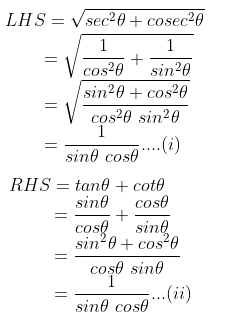From (i) and (ii),

LHS = RHS

Hence proved.

OR

(b) The thickness of a hemispherical bowl is 0.25 cm. The inner radius of the bowl is 5 cm. Find the outer curved surface area of the bowl. (take π = 22/7)

Solution:

Given,

Inner radius = r = 5 cm

Thickness of the bowl = 0.25 cm

Outer radius = R = r + thickness = 5 + 0.25 = 5.25 cm

Outer curved surface area = 2πR2

= 2 × (22/7) × 5.25 × 5.25

= 173.25 cm2

SECTION – III

31. Use venn diagrams to verify De Morgan’s law of complementation (A ⋃ B)’ = A’ ⋂ B’.

Solution: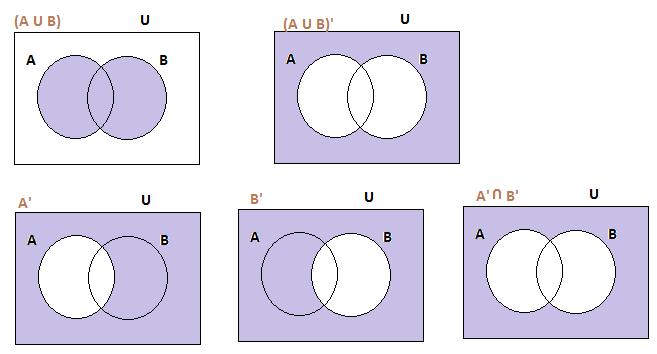Therefore, (A ⋃ B)’ = A’ ⋂ B’

32. Let A = {4, 6, 8, 10} and {3, 4, 5, 6, 7} and f : A → B be defined by f(x) = (1/2)x + 1, then represent f by:

(i) an arrow diagram

(ii) a set of ordered pairs and

(iii) a table

Solution:

Given,

f(x) = (½)x + 1

f(4) = (½) × 4 + 1 = 2 + 1 = 3

f(6) = (½) × 6 + 1 = 3 + 1 = 4

f(8) = (½) × 8 + 1 = 4 + 1 = 5

f(10) = (½) × 10 + 1 = 5 + 1 = 6

(i) An arrow diagram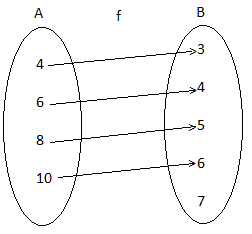(ii) The set of ordered pairs are f = {(4, 3), (6, 4), (8, 5), (10, 6)}

(iii) Table

 x 4 6 8 10 f(x) 3 4 5 6

33. Find the sum of the first n terms of the series 6 + 66 + 666 + …..

Solution:

Given,

6 + 66 + 666 + …..

Sum of first n terms

Sn = 6 + 66 + 666 + ….. + (n terms)

Sn = 6(1 + 11 + 111 + …. + n terms)

= (6/9) [9 + 99 + 999 + … + n terms]

= (6/9) [(10 – 1) + (100 – 1) + (1000 – 1) + …. + (10n – 1)]

= (6/9) [(10 + 102 + 103 + … + 10n) – (1 + 1 + 1 + … + n terms)]

= (6/9) {[10(10n – 1)/ (10 – 1)] – n}

= (6/9) {[10(10n – 1)/9] – n}

= (60/81) (10n – 1) – 6n/9

= (20/27) (10n – 1) – 2n/3

34. The sum of four consecutive terms of an AP is 20 and the sum of their squares is 120. Find those numbers.

Solution:

Let a – 3d, a – d, a + d, a + 3d be the four consecutive terms of an AP.

According to the given,

a – 3d + a – d + a + d + a + 3d = 20

4a = 20

a = 20/4 = 5

Also,

(a – 3d)2 + (a – d)2 + (a + d)2 + (a + 3d)2 = 120

a2 + 9d2 – 6ad + a2 + d2 – 2ad + a2 + d2 + 2ad + a2 + 9d2 + 6ad = 120

4a2 + 20d2 = 120

4(5)2 + 20d2 = 120

20d2 = 120 – 100

d2 = 20/20

d2 = 1

d = ±1

If a = 5 and d = -1, then AP is 8, 6, 4, 2

If a = 5 and d = 1, then AP is 2, 4, 6, 8

35. Factorize: x3 – 7x + 6

Solution:

x3 – 7x + 6 = (x3 – x2) + (x2 – 7x + 6)

= (x3 – x2) + (x2 – 7x + 6)

= (x3 – x2) + (x2 – 6x – x + 6)

= x2(x – 1) + (x – 1)(x – 6)

= (x – 1)(x2 + x – 6)

= (x – 1)(x2 + 3x – 2x – 6)

= (x – 1)(x – 2)(x + 3)

36. Find the square root of 4 + 25x2 – 12x – 24x3 + 16x4

Solution:

Given,

4 + 25x2 – 12x – 24x3 + 16x4

= 16x4 – 24x3 + 25x2 – 12x + 4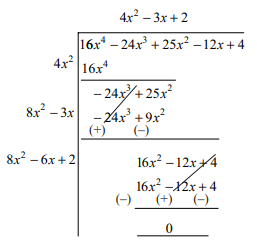Therefore, the square root of the polynomial = |4x2 – 3x + 2|

37.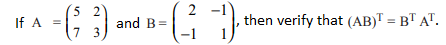Solution:

Given,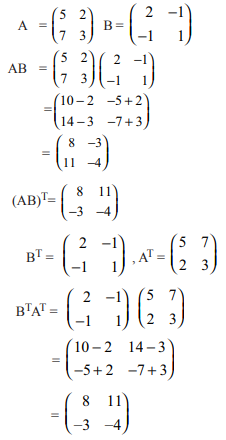Therefore, (AB)T = BTAT

38. Prove that (0, 5), (-2, -2), (5, 0) and (7, 7) are the vertices of a rhombus.

Solution:

Let the given vertices be A(0, 5), B(-2, -2), C(5, 0) and D(7, 7).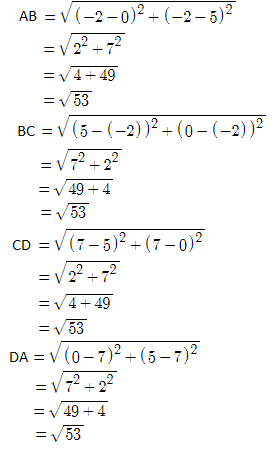AB = BC = CD = DA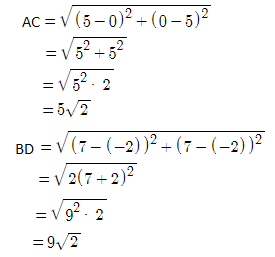Diagonal AC ≠ Diagonal BD

Hence, the given vertices form a rhombus.

39. If all sides of a parallelogram touch a circle, show that the parallelogram is a rhombus.

Solution:

Given: Sides AB,BC,CD and DA of a parallelogram ABCD touch a circle at P,Q,R and S respectively.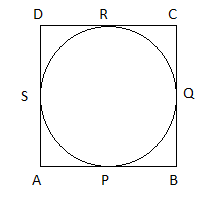To prove: Parallelogram ABCD is a rhombus.

Proof:

We know that the tangents drawn from an external point to a circle are equal.

AP = AS….(i) (tangents from point A)

BP = BQ….(ii) (tangents from point B)

CR = CQ….(iii) (tangents from point C)

DR = DS….(iv) (tangents from point D)

Adding (i), (ii), (iii), and (iv),

AP + BP + CR + DR = AS + BQ + CQ + DS

(AP + BP) + (CR + DR) = (AS + DS) + (BQ + CQ)

AB + CD = AD + BC

AB + AB = AD + AD (opposite sides of a parallelogram are equal]

Also, AB = CD and AD = BC

AB = BC = CD = DA

Hence, the parallelogram ABCD is a rhombus.

40. If tan θ + sin θ = m, tan θ – sin θ = n and m ≠ n, then show that m2 – n2 = 4√mn.

Solution:

Given,

tan θ + sin θ = m

tan θ – sin θ = n

m2 – n2 = (tan θ + sin θ)2 – (tan θ – sin θ)2

= tan2θ + sin2θ + 2 tan θ sin θ – tan2θ – sin2θ + 2 tan θ sin θ

= 4 tan θ sin θ….(i)

mn = (tan θ + sin θ)(tan θ – sin θ)

= tan2θ – sin2θ

= (sin2θ/cos2θ) – sin2θ

= sin2θ [(1 – cos2θ)/cos2θ]

= sin2θ (sin2θ/cos2θ)

= sin2θ tan2θ

⇒ √mn = tan θ sin θ ….(ii)

From (i) and (ii),

m2 – n2 = 4√mn

41. A sector containing an angle of 120° is cut off a circle of radius 21 cm and folded into a cone by joining the radii. Find the curved surface area of the cone. (π = 22/7)

Solution:

Given,

Radius of the sector = R = 21 cm

Angle of the sector = θ = 120°

Let r be the radius and l be the slant height of the cone.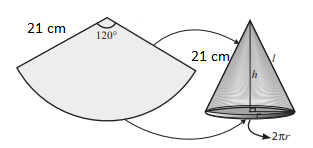Curved surface area of the cone = Area of the sector

= (θ/360°) × πR2

= (120°/360°) × (22/7) × 21 × 21

= 22 × 21

= 462 cm2

42. An iron right circular cone of diameter 8 cm and height 12 cm is melted and recast into spherical lead shots each of radius 4 mm. How many lead shots can be made?

Solution:

Given,

Diameter of cone = 8 cm

Radius of cone = R = 4 cm

Height of the cone = h = 12 cm

Radius of spherical shot = 4 mm = 0.4 cm

Number of spherical lead shots = Volume of cone/ Volume of one spherical shot

= [(1/3) πR2h]/ [(4/3) πr3]

= (4 × 4 × 12)/ (4 × 0.4 × 0.4 × 0.4)

= 750

Hence, the required number of lead shots are 750.

43. The following table shows the marks obtained by 48 students in a quiz competition in mathematics. Calculate the standard deviation.

 Data x 6 7 8 9 10 11 12 Frequency f 3 6 9 13 8 5 4

Solution:

 x f fx d = x – 9 d2 fd2 6 3 18 -3 9 27 7 6 42 -2 4 24 8 9 72 -1 1 9 9 13 117 0 0 0 10 8 80 1 1 8 11 5 55 2 4 20 12 4 48 3 9 36 ∑f = 48 ∑fx = 432 ∑d = 0 ∑fd2 = 124

Arithmetic mean = ∑fx/∑f = 432/48 = 9

Standard deviation = √(∑fd2/∑f)

= √(124/48)

= √2.58

= 1.61 (approx)

44. The probability that a new car will get an award for its design is 0.25, the probability that it will get an award for efficient use of fuel is 0.35 and the probability that it will get both the awards is 0.15. Find the probability that:

(i) it will get at least one of the two awards

(ii) it will get only one of the awards

Solution:

Let P(A) be the probability of winning the award for design and P(B) be the probability of getting an award for efficient use of fuel.

P(A) = 0.25

P(B) = 0.35

P(A ∩B) = 0.15

(i) Probability that it will get at least one of the two awards

P(A U B) = P(A) + P(B) – P(A ∩ B)

= 0.25 + 0.35 – 0.15

= 0.45

(ii) Probability of getting only one award = P(A) + P(B) – 2P(A ∩ B)

= 0.25 + 0.35 – 2(0.15)

= 0.3

45. (a) Find the LCM (Least Common Multiple) of the following.

x3 + y3, x3 – y3, x4 + x2y2 + y4

Solution:

x3 + y3 = (x + y)(x2 – xy + y2)

x3 – y3 = (x – y)(x2 + xy + y2)

x4 + x2y2 + y4 = (x2)2 + 2x2y2 + (y2)2 – x2y2

= (x2 + y2)2 – (xy)2

= (x2 + y2 + xy)(x2 + y2 – xy)

LCM = (x + y)(x – y)(x2 + y2 + xy)(x2 + y2 – xy)

= (x2 – y2)(x2 + y2 + xy)(x2 + y2 – xy)

OR

(b) Find the equation of the line whose gradient is 3/2 and which passes through P, where P divides the line segment joining A(-2, 6) and B(3, -4) in the ratio 2 : 3 internally.

Solution:

Given, P(x, y) divides the line segment joining A(-2, 6) and B(3, -4) in the ratio 2 : 3 internally.

Here,

(x1, y1) = (-2, 6)

(x2, y2) = (3, -4)

l : m = 2 : 3

Using section formula,

P(x, y) = [(lx2 + mx1)/ (l + m), (ly2 + my1)/ (l + m)]

= [(6 – 6)/ (2 + 3), (-8 + 18)/ (2 + 3)]

= (0/5, 10/5)

= (0, 2)

Equation of the line passing through P(0, 2) with slope 3/2 is:

y – y1 = m(x – x1)

y – 2 = (3/2)(x – 0)

y – 2 = (3/2)x

2y – 4 = 3x

⇒ 3x – 2y + 4 = 0

SECTION – IV

46. (a) Draw a circle of diameter 10 cm. From a point P, 13 cm away from its centre, draw the two tangents PA and PB to the circle and measure their lengths.

Solution:

Given,

Diameter of circle = 10 cm

Radius = 10/2 = 5 cm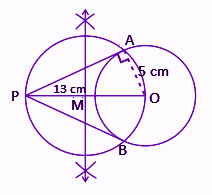PA and PB are required tangents.

Length of the tangents = 12 cm

OR

(b) Construct a ΔABC in which the base BC = 5 cm, ∠BAC = 40° and the median from A to BC is 6 cm. Also, measure the length of the altitude from A.

Solution: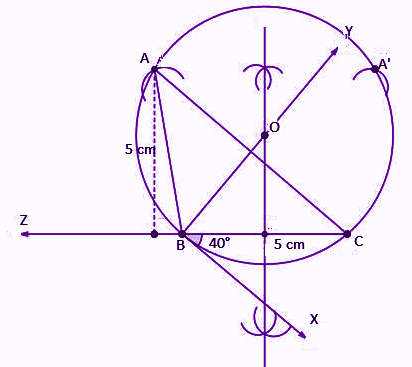47. (a) Draw the graph of y = x2 + 2x – 3 and hence find the roots x2 – x – 6 = 0.

Solution:

Given,

y = x2 + 2x – 3

 x -3 -2 -1 0 1 2 3 y 0 -3 -4 -3 0 5 12

Now, subtracting x2 – x – 6 = 0 from y = x2 + 2x – 3,

y – 0 = x2 + 2x – 3 – (x2 – x – 6)

y = 3x + 3

 x -2 -1 0 1 2 3 y -3 0 3 6 9 12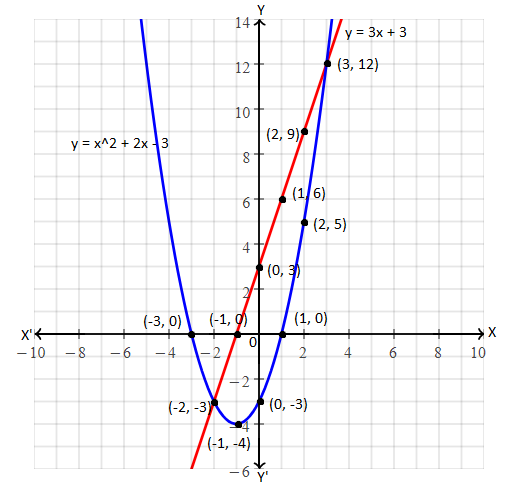The graph of given equations intersect each other at (-2, -3) and (3, 12).

Hence, the solution set is (-2, -3) and (3, 12).

OR

(b) A bank gives 10% SI (simple interest) on deposits for senior citizens. Draw the graph for the relation between the sum deposited and the interest earned for one year. Hence, find:

(i) the interest on the deposit of Rs. 650

(ii) The amount to be deposited to earn an interest of Rs. 45

Solution:

Simple interest = PTR/100

Given, R = 10%

SI = (P × T × 10)/100 = PT/100

SI for 1 year = P/100

Let us take different values of P as shown below.

 P 100 200 300 400 500 600 700 800 SI 10 20 30 40 50 60 70 80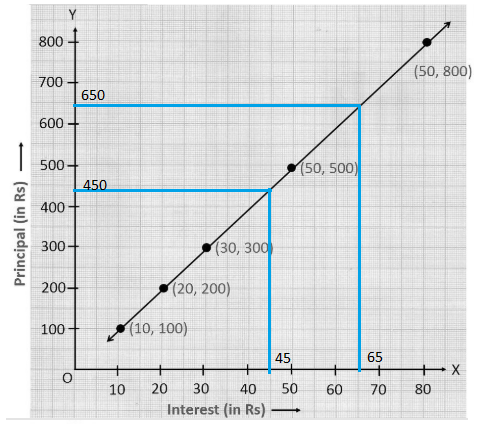(i) Simple interest earned on a deposit of Rs. 650 = Rs. 65

(ii) The amount to be deposited to earn an interest of Rs. 45 = Rs. 450